ROOT   Reference Guide
Searching...
No Matches
TGaxis Class Reference

The axis painter class.

Instances of this class are generated by the histograms and graphs painting classes when TAxis are drawn. TGaxis is the "painter class" of TAxis. Therefore it is mainly used via TAxis, even if is some occasion it can be used directly to draw an axis which is not part of a graph or an instance. For instance to draw an extra scale on a plot.

## Basic definition

A TGaxis is defined the following way:

Double_t gridlength)
const char Option_t
Definition RtypesCore.h:66
Option_t Option_t TPoint TPoint const char GetTextMagnitude GetFillStyle GetLineColor GetLineWidth GetMarkerStyle GetTextAlign GetTextColor GetTextSize void char Point_t Rectangle_t wmin
Option_t Option_t TPoint TPoint const char GetTextMagnitude GetFillStyle GetLineColor GetLineWidth GetMarkerStyle GetTextAlign GetTextColor GetTextSize void char Point_t Rectangle_t wmax
float xmin
float ymin
float xmax
float ymax
TGaxis()
TGaxis default constructor.
Definition TGaxis.cxx:694

Where:

• xmin : X origin coordinate in user's coordinates space.
• xmax : X end axis coordinate in user's coordinates space.
• ymin : Y origin coordinate in user's coordinates space.
• ymax : Y end axis coordinate in user's coordinates space.
• wmin : Lowest value for the tick mark labels written on the axis.
• wmax : Highest value for the tick mark labels written on the axis.
• ndiv : Number of divisions.
• ndiv=N1 + 100*N2 + 10000*N3
• N1=number of 1st divisions.
• N2=number of 2nd divisions.
• N3=number of 3rd divisions. e.g.:
• ndiv=0 --> no tick marks.
• ndiv=2 --> 2 divisions, one tick mark in the middle of the axis.
• chopt : Drawing options (see below).
• gridlength: grid length on main tick marks.

The example below generates various kind of axis.

void gaxis(){
auto c1 = new TCanvas("c1","Examples of TGaxis",10,10,700,500);
c1->Range(-10,-1,10,1);
auto axis1 = new TGaxis(-4.5,-0.2,5.5,-0.2,-6,8,510,"");
axis1->Draw();
auto axis2 = new TGaxis(-4.5,0.2,5.5,0.2,0.001,10000,510,"G");
axis2->Draw();
auto axis3 = new TGaxis(-9,-0.8,-9,0.8,-8,8,50510,"");
axis3->SetTitle("axis3");
axis3->SetTitleOffset(0.5);
axis3->Draw();
auto axis4 = new TGaxis(-7,-0.8,-7,0.8,1,10000,50510,"G");
axis4->SetTitle("axis4");
axis4->Draw();
auto axis5 = new TGaxis(-4.5,-0.6,5.5,-0.6,1.2,1.32,80506,"-+");
axis5->SetLabelSize(0.03);
axis5->SetTextFont(72);
axis5->Draw();
auto axis6 = new TGaxis(-4.5,0.5,5.5,0.5,100,900,50510,"-");
axis6->Draw();
auto axis7 = new TGaxis(-5.5,0.85,5.5,0.85,0,4.3e-6,510,"");
axis7->Draw();
auto axis8 = new TGaxis(8,-0.8,8,0.8,0,9000,50510,"+L");
axis8->Draw();
// One can make a vertical axis going top->bottom. However the two x values should be
// slightly different to avoid labels overlapping.
auto axis9 = new TGaxis(6.5,0.8,6.499,-0.8,0,90,50510,"-");
axis9->Draw();
}
The Canvas class.
Definition TCanvas.h:23
return c1
Definition legend1.C:41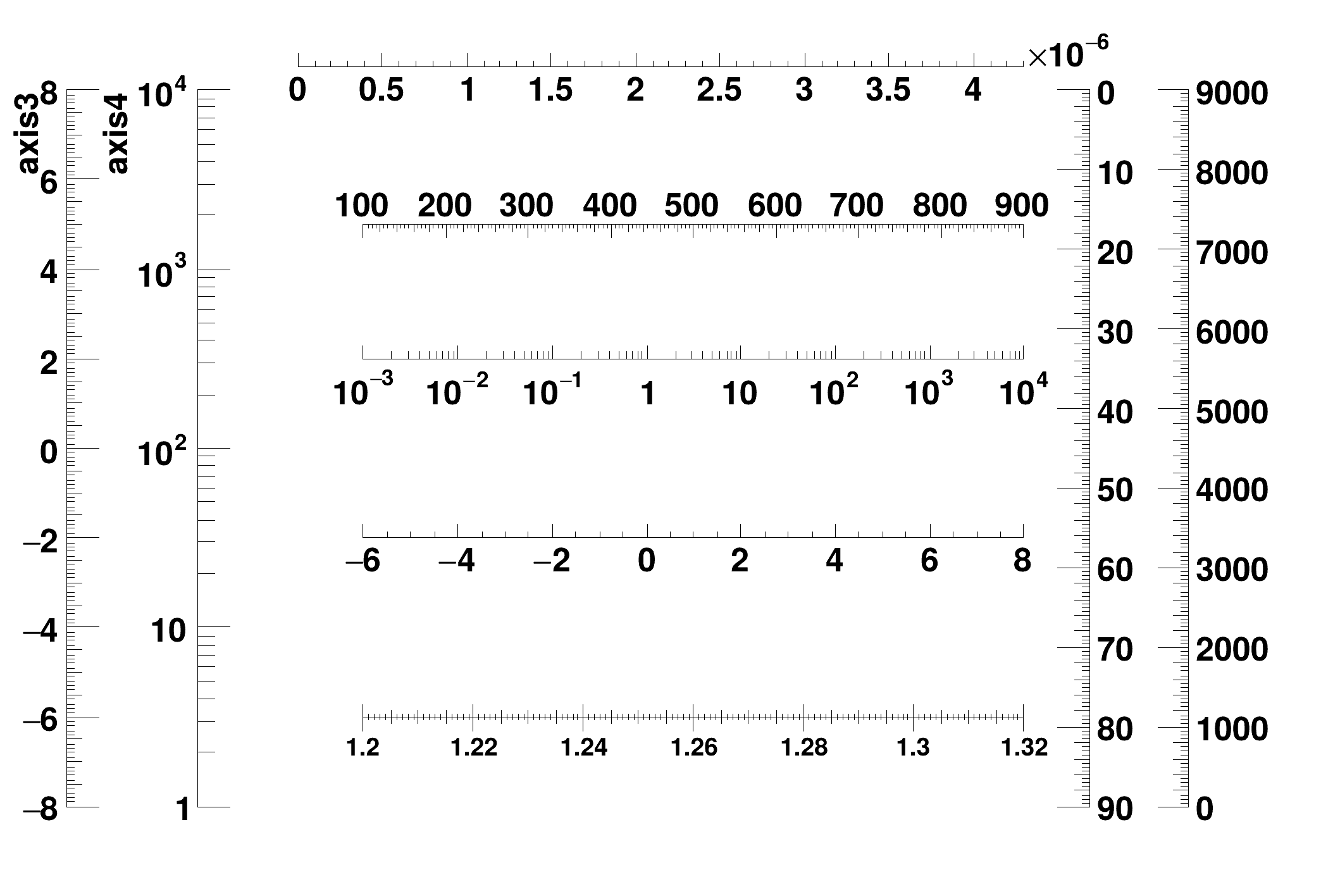## Definition with a function

Instead of the wmin,wmax arguments of the normal definition, the name of a TF1 function can be specified. This function will be used to map the user coordinates to the axis values and ticks.

A TGaxis is defined the following way:

const char *func, Int_t ndiv, Option_t *chopt,
Double_t gridlength)

Where:

• xmin : X origin coordinate in user's coordinates space.
• xmax : X end axis coordinate in user's coordinates space.
• ymin : Y origin coordinate in user's coordinates space.
• ymax : Y end axis coordinate in user's coordinates space.
• func : function defining axis labels and tick marks.
• ndiv : Number of divisions.
• ndiv=N1 + 100*N2 + 10000*N3
• N1=number of 1st divisions.
• N2=number of 2nd divisions.
• N3=number of 3rd divisions. e.g.:
• ndiv=0 --> no tick marks.
• ndiv=2 --> 2 divisions, one tick mark in the middle of the axis.
• chopt : Drawing options (see below).
• gridlength: grid length on main tick marks.

It should be noted that func is not defined in the user's coordinate space, but in the new TGaxis space. If x is the original axis, w the new axis, and w = f(x) (for example, f is a calibration function converting ADC channels x to energy w), then func must be supplied as f^{-1}(w).

Examples:

{
TCanvas *c2 = new TCanvas("c2","c2",10,10,700,500);
TF1 *f1=new TF1("f1","-x",-10,10);
TGaxis *A1 = new TGaxis(0,2,10,2,"f1",510,"-");
A1->SetTitle("axis with decreasing values");
A1->Draw();
TF1 *f2=new TF1("f2","exp(x)",0,2);
TGaxis *A2 = new TGaxis(1,1,9,1,"f2");
A2->SetTitle("exponential axis");
A2->SetLabelSize(0.03);
A2->SetTitleSize(0.03);
A2->SetTitleOffset(1.2);
A2->Draw();
TF1 *f3=new TF1("f3","log10(x)",1,1000);
TGaxis *A3 = new TGaxis(2,-2,2,0,"f3",505,"");
A3->SetTitle("logarithmic axis");
A3->SetLabelSize(0.02);
A3->SetTitleSize(0.03);
A3->SetTitleOffset(0.); // Axis title automatically placed
A3->Draw();
}
1-Dim function class
Definition TF1.h:213
The axis painter class.
Definition TGaxis.h:24
void SetTitleOffset(Float_t titleoffset=1)
Definition TGaxis.h:128
void SetTitleSize(Float_t titlesize)
Definition TGaxis.h:129
void SetLabelSize(Float_t labelsize)
Definition TGaxis.h:107
virtual void SetTitle(const char *title="")
virtual void Draw(Option_t *option="")
Default Draw method for all objects.
Definition TObject.cxx:274
TF1 * f1
Definition legend1.C:11
return c2
Definition legend2.C:14Note that this functionality has some limitations and does not follow all the TGaxis setting. In particular the number of divisions or the maximum number digits do not apply.

## Logarithmic axis

By default axis are linear. To define a TGaxis as logarithmic, it is enough to create it with the option "G".

When plotting an histogram or a graph the logarithmic scale can be set using:

• gPad->SetLogx(1); set the logarithmic scale on the X axis
• gPad->SetLogy(1); set the logarithmic scale on the Y axis

When the SetMoreLogLabels() method is called more labels are drawn when in logarithmic scale and there is a small number of decades (less than 3).

## Blank axis

To draw only the axis tick marks without the axis body, it is enough to specify the option "B". It useful to superpose axis.

## Arrow on axis

Since
ROOT version 6.27/01:

To draw an arrow at the end of the axis use the option ">". To draw it at the beginning of the axis use the option "<". To draw it on both ends use "<>".

{
auto c = new TCanvas("c","c",0,0,500,500);
c->Range(-11,-11,11,11);
auto f2 = new TF1("x2","x*x",-10,10);
f2->Draw("same");
auto f3 = new TF1("x3","x*x*x",-10,10);
f3->Draw("same");
// Draw the axis with arrows
auto ox = new TGaxis(-10,0,10,0,-10.,10.,510,"+-S>");
ox->SetTickSize(0.009);
ox->SetLabelFont(42);
ox->SetLabelSize(0.025);
ox->Draw();
auto oy = new TGaxis(0,-10,0,10,-10,10,510,"+-S>");
oy->SetTickSize(0.009);
oy->SetLabelFont(42);
oy->SetLabelSize(0.025);
oy->Draw();
}
#define c(i)
Definition RSha256.hxx:101
@ kRed
Definition Rtypes.h:66
@ kBlue
Definition Rtypes.h:66
virtual void SetLineColor(Color_t lcolor)
Set the line color.
Definition TAttLine.h:40
void Draw(Option_t *option="") override
Draw this function with its current attributes.
Definition TF1.cxx:1334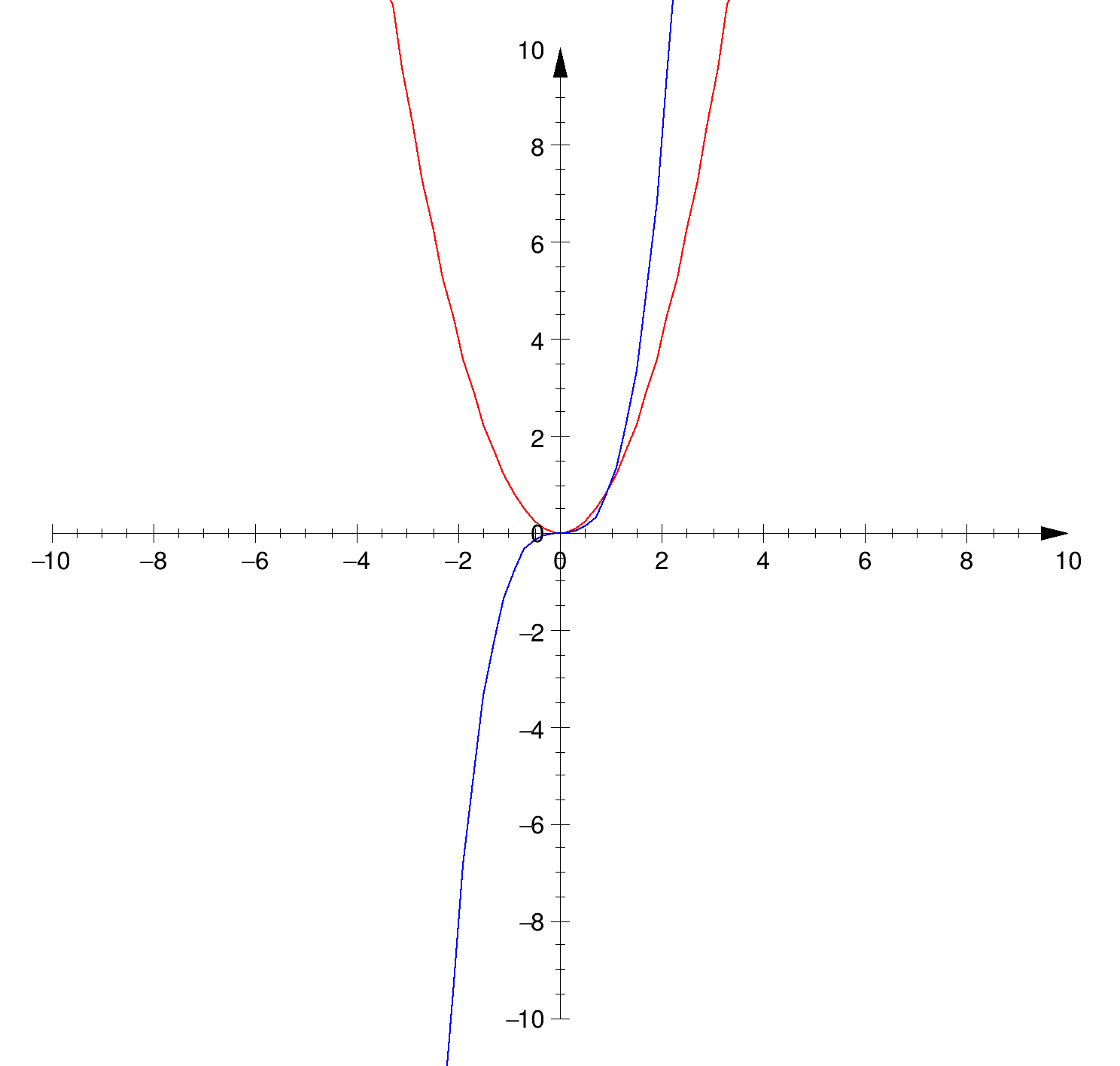## Tick marks' orientation

By default tick marks are drawn on the positive side of the axis, except for vertical axis for which the default is negative. The chop parameter allows to control the tick marks orientation:

• chopt = "+": tick marks are drawn on Positive side. (default)
• chopt ="-": tick mark are drawn on the negative side.
• chopt = "+-": tick marks are drawn on both sides of the axis.
• chopt = "U": Unlabelled axis, default is labeled.

## Tick marks' size

By default, tick marks have a length equal to 3 per cent of the axis length. When the option "S" is specified, the length of the tick marks is equal to fTickSize*axis_length, where fTickSize may be set via TGaxis::SetTickSize.

When plotting an histogram h the tick marks size can be changed using:

• h->GetXaxis()->SetTickLength(0.02); set the tick length for the X axis
• gStyle->SetTickLength(0.02,"x"); set the tick length for the X axis of all histograms drawn after this instruction.

A good way to remove tick marks on an axis is to set the tick length to 0: h->GetXaxis()->SetTickLength(0.);

## Labels' positioning

Labels are normally drawn on side opposite to tick marks. However the option "=" allows to draw them on the same side. The distance between the labels and the axis body can be changed with SetLabelOffset.

## Labels' orientation

By default axis labels are drawn parallel to the axis. However if the axis is vertical then are drawn perpendicular to the axis.

## Labels' position on tick marks

By default axis labels are centered on tick marks. However, for vertical axis, they are right adjusted. The chop parameter allows to control the labels' position on tick marks:

• chopt = "R": labels are Right adjusted on tick mark.(default is centered)
• chopt = "L": labels are Left adjusted on tick mark.
• chopt = "C": labels are Centered on tick mark.
• chopt = "M": In the Middle of the divisions.

## Labels' format

Blank characters are stripped, and then the label is correctly aligned. the dot, if last character of the string, is also stripped, unless the option "." (a dot, or period) is specified. if SetDecimals(kTRUE) has been called all labels have the same number of decimals after the "." The same is true if gStyle->SetStripDecimals(kFALSE) has been called.

In the following, we have some parameters, like tick marks length and characters height (in percentage of the length of the axis (user's coordinates)) The default values are as follows:

• Primary tick marks: 3.0 %
• Secondary tick marks: 1.5 %
• Third order tick marks: .75 %
• Characters height for labels: 4%
• Labels offset: 1.0 %

By default, an exponent of the form 10^N is used when the label values are either all very small or very large. One can disable the exponent by calling axis.SetNoExponent(kTRUE).

TGaxis::SetExponentOffset(Float_t xoff, Float_t yoff, Option_t *axis) is static function to set X and Y offset of the axis 10^n notation. It is in % of the pad size. It can be negative. axis specifies which axis ("x" or/and "y"), default is "x" if axis = "xz" set the two axes

## Alphanumeric labels

Axis labels can be any alphanumeric character strings. Such axis can be produced only with histograms because the labels'definition is stored in TAxis. The following example demonstrates how to create such labels.

TCanvas *hlabels2()
{
const Int_t nx = 12;
const Int_t ny = 20;
const char *month[nx] = {"January","February","March","April","May",
"June","July","August","September","October","November",
"December"};
const char *people[ny] = {"Jean","Pierre","Marie","Odile","Sebastien",
"Fons","Rene","Nicolas","Xavier","Greg","Bjarne","Anton",
"Otto","Eddy","Peter","Pasha","Philippe","Suzanne","Jeff",
"Valery"};
TCanvas *c1 = new TCanvas("c1","demo bin labels",10,10,600,600);
c1->SetGrid();
c1->SetLeftMargin(0.15);
c1->SetBottomMargin(0.15);
TH2F *h = new TH2F("h","test",3,0,3,2,0,2);
h->SetCanExtend(TH1::kAllAxes);
h->SetStats(0);
for (Int_t i=0;i<15000;i++) {
Int_t rx = gRandom->Rndm()*nx;
Int_t ry = gRandom->Rndm()*ny;
h->Fill(people[ry],month[rx],1);
}
h->LabelsDeflate("X");
h->LabelsDeflate("Y");
h->LabelsOption("v");
h->Draw("text");
TPaveText *pt = new TPaveText(0.6,0.85,0.98,0.98,"brNDC");
pt->AddText(" \"a\" to sort by alphabetic order");
pt->AddText(" \">\" to sort by decreasing values");
pt->AddText(" \"<\" to sort by increasing values");
pt->Draw();
return c1;
}
#define h(i)
Definition RSha256.hxx:106
int Int_t
Definition RtypesCore.h:45
R__EXTERN TRandom * gRandom
Definition TRandom.h:62
virtual void SetFillColor(Color_t fcolor)
Set the fill area color.
Definition TAttFill.h:37
virtual void SetTextAlign(Short_t align=11)
Set the text alignment.
Definition TAttText.h:42
@ kAllAxes
Definition TH1.h:75
2-D histogram with a float per channel (see TH1 documentation)}
Definition TH2.h:258
A Pave (see TPave) with text, lines or/and boxes inside.
Definition TPaveText.h:21
virtual TText * AddText(Double_t x1, Double_t y1, const char *label)
Add a new Text line to this pavetext at given coordinates.
void Draw(Option_t *option="") override
Draw this pavetext with its current attributes.
virtual void SetSeed(ULong_t seed=0)
Set the random generator seed.
Definition TRandom.cxx:608
Double_t Rndm() override
Machine independent random number generator.
Definition TRandom.cxx:552
TPaveText * pt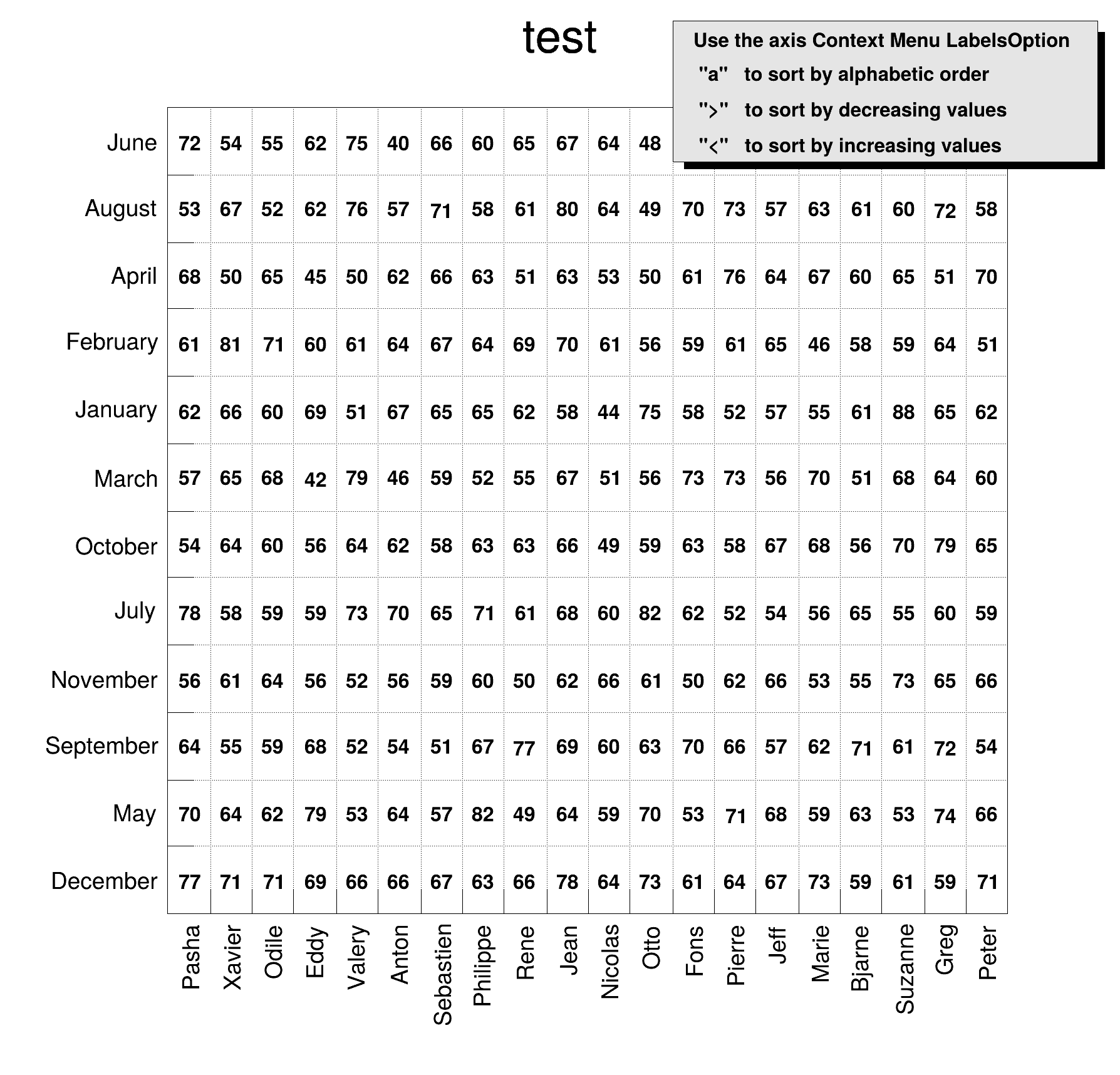Because the alphanumeric labels are usually longer that the numeric labels, their size is by default equal to 0.66666 * the_numeric_labels_size.

## Changing axis labels

Since
ROOT version 6.07/07:

After an axis has been created, TGaxis::ChangeLabel allows to define new text attributes for a given label. A fine tuning of the labels can be done. All the attributes can be changed as well as the text label itself.

When plotting an histogram or a graph the labels can be changed like in the following example which shows a way to produce $$\pi$$-axis :

{
TF1* f = new TF1("f","TMath::Cos(x/TMath::Pi())", -pi, pi);
TAxis* a = f->GetXaxis();
a->SetNdivisions(-502);
a->ChangeLabel(1,-1,-1,-1,-1,-1,"-#pi");
a->ChangeLabel(-1,-1,-1,-1,-1,-1,"#pi");
f->Draw();
}
#define f(i)
Definition RSha256.hxx:104
#define a(i)
Definition RSha256.hxx:99
virtual void SetNdivisions(Int_t n=510, Bool_t optim=kTRUE)
Set the number of divisions for this axis.
Definition TAttAxis.cxx:233
Class to manage histogram axis.
Definition TAxis.h:31
constexpr Double_t Pi()
Definition TMath.h:37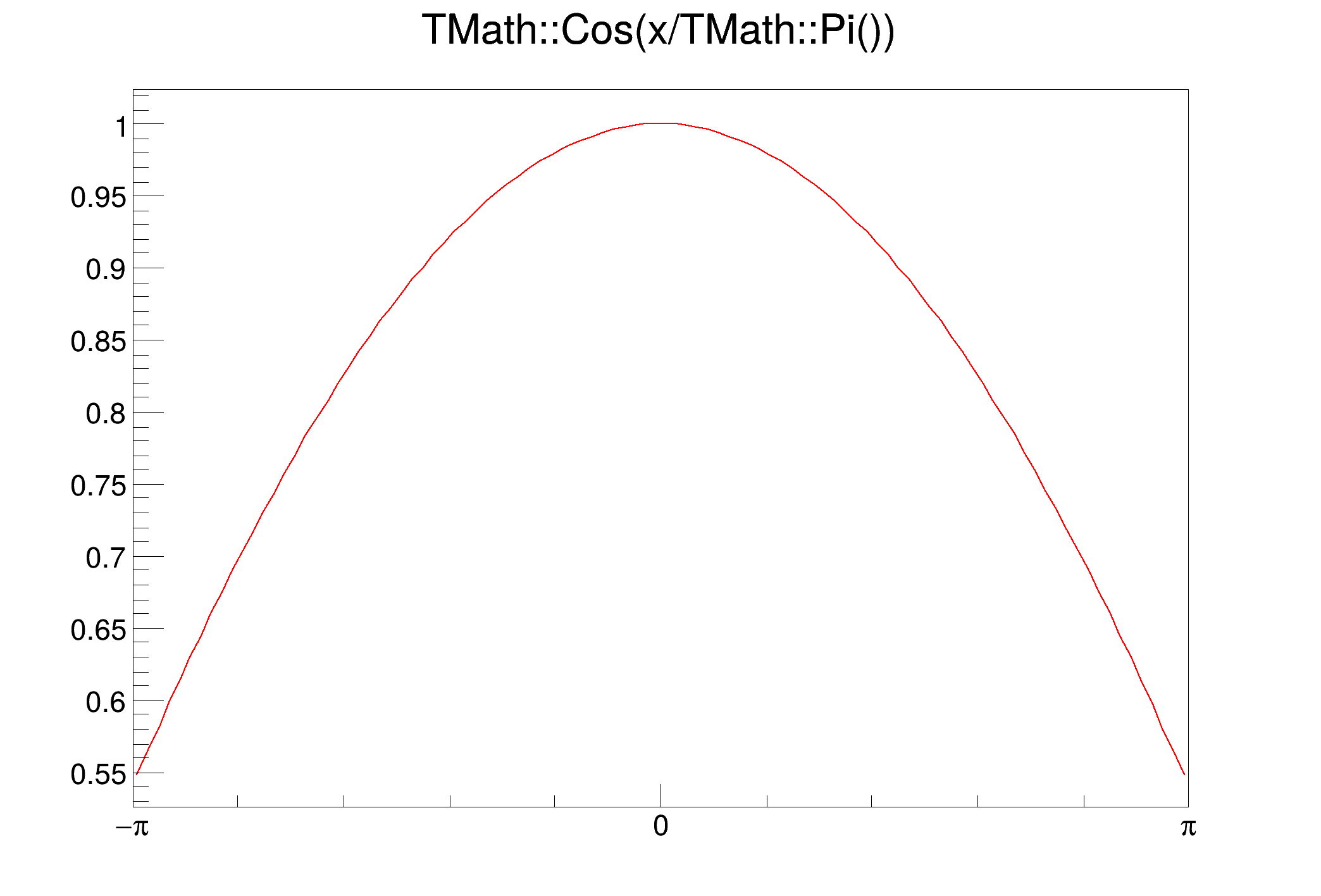## Number of divisions optimisation

By default the number of divisions on axis is optimised to show a coherent labelling of the main tick marks. The number of division (ndiv) is a composite integer given by:

ndiv = N1 + 100*N2 + 10000*N3

• N1 = number of 1st divisions.
• N2 = number of 2nd divisions.
• N3 = number of 3rd divisions.

by default the value of N1, N2 and N3 are maximum values. After optimisation the real number of divisions will be smaller or equal to these value. If one wants to bypass the optimisation, the option "N" should be given when the TGaxis is created. The option "I" also act on the number of division as it will force an integer labelling of the axis.

On an histogram pointer h the number of divisions can be set in different ways:.

• Directly on the histogram. The following will set the number of division to 510 on the X axis of h. To avoid optimization the number of divisions should be negative (ie: -510);
h->SetNdivisions(510, "X");
• On the axis itself:
h->GetXaxis()->SetNdivisions(510, kTRUE);
const Bool_t kTRUE
Definition RtypesCore.h:100

The first parameter is the number of division. If it is negative of if the second parameter is kFALSE then the number of divisions is not optimised. And other signature is also allowed:

h->GetXaxis()->SetNdivisions(10, 5, 0, kTRUE);

## Maximum Number of Digits for the axis labels

The static method TGaxis::SetMaxDigits sets the maximum number of digits permitted for the axis labels above which the notation with 10^N is used. For example, to accept 6 digits number like 900000 on an axis call TGaxis::SetMaxDigits(6). The default value is 5. fgMaxDigits must be greater than 0. Warning: even when called on a particular TGaxis* instance, this static function changes globally the number of digits for all axes (X, Y, ...) in the canvas. If you want to change the maximum number of digits N only of the current TGaxis*, and not all the others, use axis->SetNdivisions(N*1000000 + (axis->GetNdiv()%1000000)) instead of axis->SetMaxDigits(N).

## Optional grid

The option "W" allows to draw a grid on the primary tick marks. In case of a log axis, the grid is only drawn for the primary tick marks if the number of secondary and tertiary divisions is 0. SetGridLength() allows to define the length of the grid.

When plotting an histogram or a graph the grid can be set ON or OFF using:

• gPad->SetGridy(1); set the grid on the X axis
• gPad->SetGridx(1); set the grid on the Y axis
• gPad->SetGrid(1,1); set the grid on both axis.

## Time axis

Histograms' axis can be defined as "time axis". To do that it is enough to activate the TAxis::SetTimeDisplay attribute on a given axis. If h is an histogram, it is done the following way:

.cpp
h->GetXaxis()->SetTimeDisplay(1); // The X axis is a time axis

Two parameters can be adjusted in order to define time axis:

### The time format:

Defines the format of the labels along the time axis. It can be changed using the TAxis TAxis::SetTimeFormat. The time format is the one used by the C function strftime(). It's a string containing the following formatting characters:

• for date :
• a abbreviated weekday name
• b abbreviated month name
• d day of the month (01-31)
• m month (01-12)
• y year without century
• Y year with century
• for time :
• H hour (24-hour clock)
• I hour (12-hour clock)
• p local equivalent of AM or PM
• M minute (00-59)
• S seconds (00-61)
• %% %

The other characters are output as is. For example to have a format like dd/mm/yyyy one should do:

.cpp
h->GetXaxis()->SetTimeFormat("%d\/%m\/%Y");

### The time offset:

This is a time in seconds in the UNIX standard UTC format (this is an universal time, not the local time), defining the starting date of an histogram axis. This date should be greater than 01/01/95 and is given in seconds. There are three ways to define the time offset:

#### By setting the global default time offset:

.cpp
TDatime da(2003,02,28,12,00,00);
gStyle->SetTimeOffset(da.Convert());
R__EXTERN TStyle * gStyle
Definition TStyle.h:433
This class stores the date and time with a precision of one second in an unsigned 32 bit word (950130...
Definition TDatime.h:37
void SetTimeOffset(Double_t toffset)
Change the time offset for time plotting.
Definition TStyle.cxx:1909

If no time offset is defined for a particular axis, the default time offset will be used. In the example above, notice the usage of TDateTime to translate an explicit date into the time in seconds required by TAxis::SetTimeFormat.

#### By setting a time offset to a particular axis:

.cpp
TDatime dh(2001,09,23,15,00,00);
h->GetXaxis()->SetTimeOffset(dh.Convert());

#### Together with the time format using TAxis::SetTimeFormat:

The time offset can be specified using the control character F after the normal time format. F is followed by the date in the format: yyyy-mm-dd hh:mm:ss.

Example:

.cpp
h->GetXaxis()->SetTimeFormat("%d\/%m\/%y%F2000-02-28 13:00:01");

Notice that this date format is the same used by the TDateString function AsSQLString. If needed, this function can be used to translate a time in seconds into a character string which can be appended after F. If the time format is not specified (before F), the automatic one will be used.

If a time axis has no specified time offset, the global time offset will be stored in the axis data structure.

The following example illustrates the various possibilities.

{
gStyle->SetTitleH(0.08);
TDatime da(2003,2,28,12,00,00);
gStyle->SetTimeOffset(da.Convert());
auto ct = new TCanvas("ct","Time on axis",0,0,600,600);
ct->Divide(1,3);
auto ht1 = new TH1F("ht1","ht1",30000,0.,200000.);
auto ht2 = new TH1F("ht2","ht2",30000,0.,200000.);
auto ht3 = new TH1F("ht3","ht3",30000,0.,200000.);
for (Int_t i=1;i<30000;i++) {
auto noise = gRandom->Gaus(0,120);
ht1->SetBinContent(i,noise);
ht2->SetBinContent(i,noise*noise);
ht3->SetBinContent(i,noise*noise*noise);
}
ct->cd(1);
ht1->GetXaxis()->SetLabelSize(0.06);
ht1->GetXaxis()->SetTimeDisplay(1);
ht1->GetXaxis()->SetTimeFormat("%d/%m/%y%F2000-02-28 13:00:01");
ht1->Draw();
ct->cd(2);
ht2->GetXaxis()->SetLabelSize(0.06);
ht2->GetXaxis()->SetTimeDisplay(1);
ht2->GetXaxis()->SetTimeFormat("%d/%m/%y");
ht2->Draw();
ct->cd(3);
ht3->GetXaxis()->SetLabelSize(0.06);
TDatime dh(2001,9,23,15,00,00);
ht3->GetXaxis()->SetTimeDisplay(1);
ht3->GetXaxis()->SetTimeOffset(dh.Convert());
ht3->Draw();
}
1-D histogram with a float per channel (see TH1 documentation)}
Definition TH1.h:577
virtual Double_t Gaus(Double_t mean=0, Double_t sigma=1)
Samples a random number from the standard Normal (Gaussian) Distribution with the given mean and sigm...
Definition TRandom.cxx:274
void SetTitleH(Float_t h=0)
Definition TStyle.h:413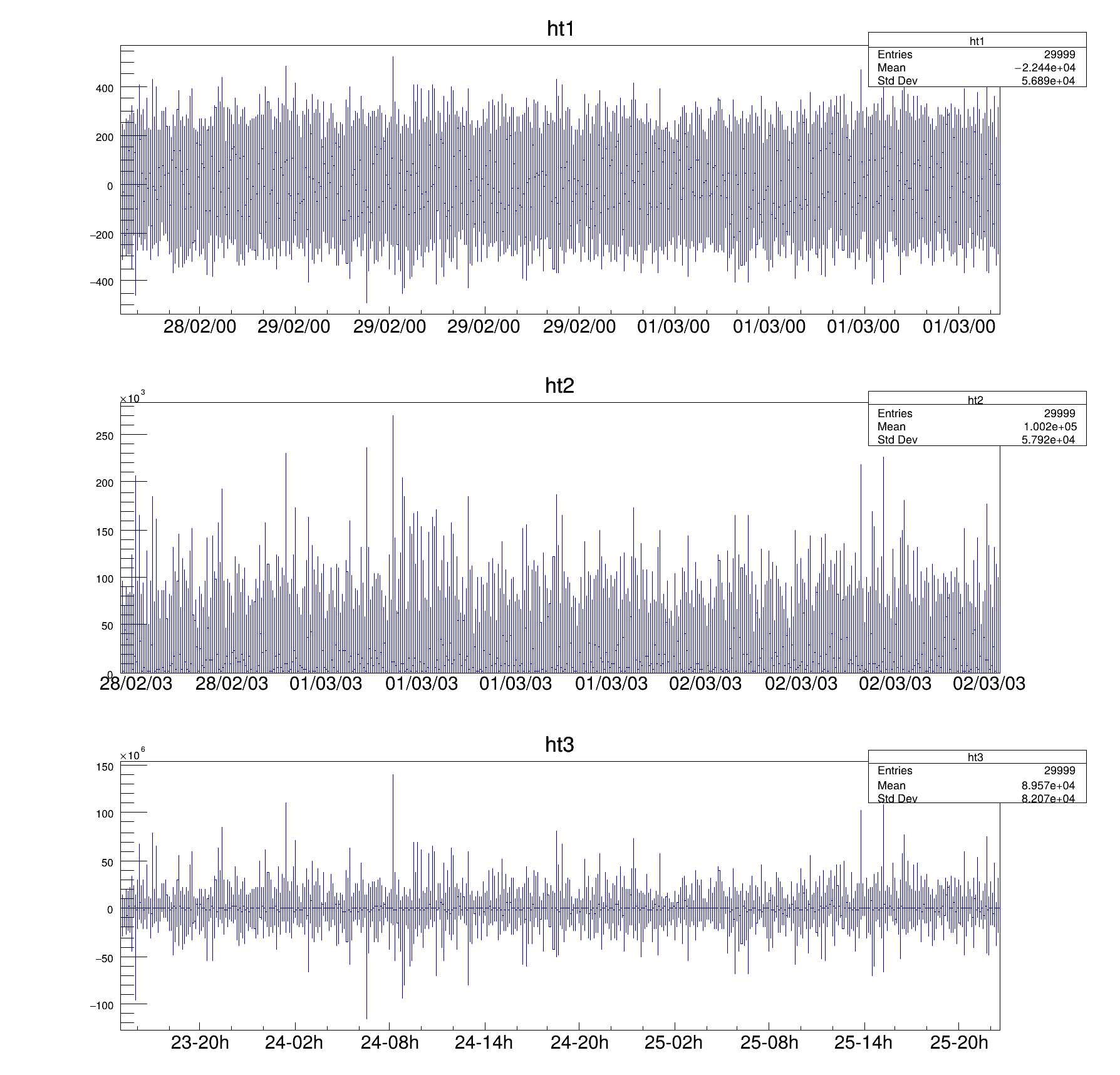The histogram limits times in seconds. If wmin and wmax are the histogram limits, the time axis will spread around the time offset value from TimeOffset+wmin to TimeOffset+wmax. Until now all the examples had a lowest value equal to 0. The following example demonstrates how to define the histogram limits relatively to the time offset value.

{
// Define the time offset as 2003, January 1st
TDatime T0(2003,1,1,0,0,0);
auto X0 = T0.Convert();
// Define the lowest histogram limit as 2002, September 23rd
TDatime T1(2002,9,23,0,0,0);
auto X1 = T1.Convert()-X0;
// Define the highest histogram limit as 2003, March 7th
TDatime T2(2003,3,7,0,0,0);
auto X2 = T2.Convert(1)-X0;
auto h1 = new TH1F("h1","test",100,X1,X2);
for (Int_t i=0;i<30000;i++) {
Double_t noise = r.Gaus(0.5*(X1+X2),0.1*(X2-X1));
h1->Fill(noise);
}
h1->GetXaxis()->SetTimeFormat("%Y/%m/%d");
h1->Draw();
}
Option_t Option_t TPoint TPoint const char GetTextMagnitude GetFillStyle GetLineColor GetLineWidth GetMarkerStyle GetTextAlign GetTextColor GetTextSize void char Point_t Rectangle_t WindowAttributes_t Float_t r
virtual void SetLabelSize(Float_t size=0.04)
Set size of axis labels.
Definition TAttAxis.cxx:203
virtual void SetTimeDisplay(Int_t value)
Definition TAxis.h:171
virtual void SetTimeFormat(const char *format="")
Change the format used for time plotting.
Definition TAxis.cxx:1151
TAxis * GetXaxis()
Definition TH1.h:322
virtual Int_t Fill(Double_t x)
Increment bin with abscissa X by 1.
Definition TH1.cxx:3341
void Draw(Option_t *option="") override
Draw this histogram with options.
Definition TH1.cxx:3063
This is the base class for the ROOT Random number generators.
Definition TRandom.h:27
TH1F * h1
Definition legend1.C:5
#define T2
Definition md5.inl:147
#define T1
Definition md5.inl:146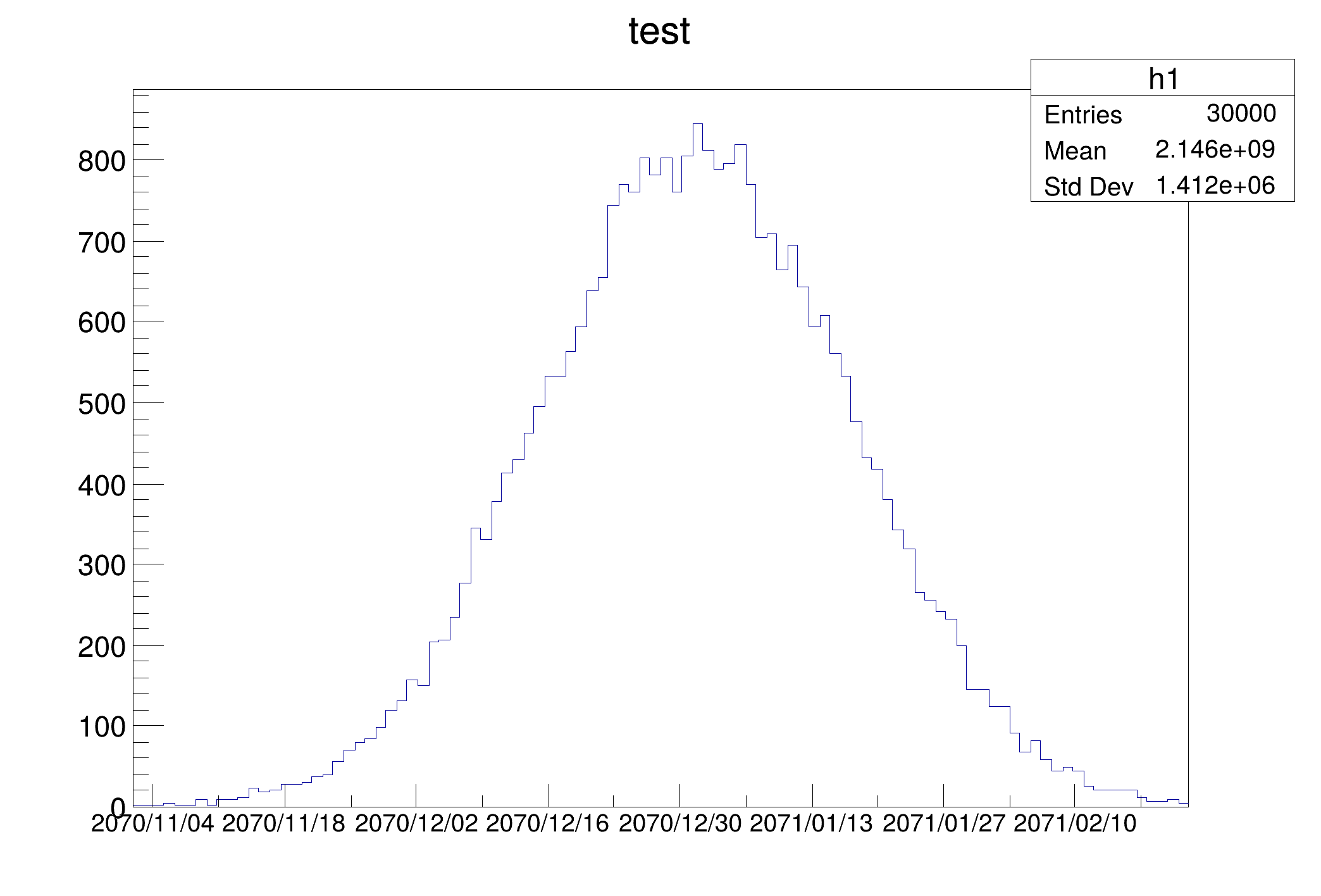Usually time axis are created automatically via histograms, but one may also want to draw a time axis outside an "histogram context". Therefore it is useful to understand how TGaxis works for such axis.

The time offset can be defined using one of the three methods described before. The time axis will spread around the time offset value. Actually it will go from TimeOffset+wmin to TimeOffset+wmax where wmin and wmax are the minimum and maximum values (in seconds) of the axis. Let's take again an example. Having defined "2003, February 28 at 12h" we would like to see the axis a day before and a day after. A TGaxis can be created the following way (a day has 86400 seconds):

.cpp
TGaxis *axis = new TGaxis(x1,y1,x2,y2,-100000,150000,2405,"t");
Option_t Option_t TPoint TPoint const char x2
Option_t Option_t TPoint TPoint const char x1
Option_t Option_t TPoint TPoint const char y2
Option_t Option_t TPoint TPoint const char y1

the t option (in lower case) means it is a "time axis". The axis goes form 100000 seconds before TimeOffset and 150000 seconds after.

So the complete macro is:

{
c1 = new TCanvas("c1","Examples of TGaxis",10,10,700,100);
c1->Range(-10,-1,10,1);
TGaxis *axis = new TGaxis(-8,0.,8,0.,-100000,150000,2405,"tS");
axis->SetLabelSize(0.2);
axis->SetTickSize(0.2);
TDatime da(2003,02,28,12,00,00);
axis->SetTimeOffset(da.Convert());
axis->SetTimeFormat("%d-%m-%Y");
axis->Draw();
return c1;
}
void SetTimeFormat(const char *tformat)
void SetTimeOffset(Double_t toffset, Option_t *option="local")
void SetTickSize(Float_t ticksize)
Definition TGaxis.h:122Thanks to the TLatex directive #splitline it is possible to write the time labels on two lines. In the previous example changing the SetTimeFormat line by

.cpp
axis->SetLabelOffset(0.15);
axis->SetTimeFormat("#splitline{%Y}{%d\/%m}");
void SetLabelOffset(Float_t labeloffset)
Definition TGaxis.h:106

will produce the following axis:The following example shows time axis on a TGraph:

{
TDatime da1(2008,02,28,15,52,00);
TDatime da2(2008,02,28,15,53,00);
double x,y;
y = 1.;
y = 2.;
x = da1.Convert();
x = da2.Convert();
TGraph mgr(2,x,y);
mgr.SetMarkerStyle(20);
mgr.Draw("apl");
mgr.GetXaxis()->SetTimeDisplay(1);
mgr.GetXaxis()->SetNdivisions(-503);
mgr.GetXaxis()->SetTimeFormat("%Y-%m-%d %H:%M");
mgr.GetXaxis()->SetTimeOffset(0,"gmt");
}
A TGraph is an object made of two arrays X and Y with npoints each.
Definition TGraph.h:41
Double_t y[n]
Definition legend1.C:17
Double_t x[n]
Definition legend1.C:17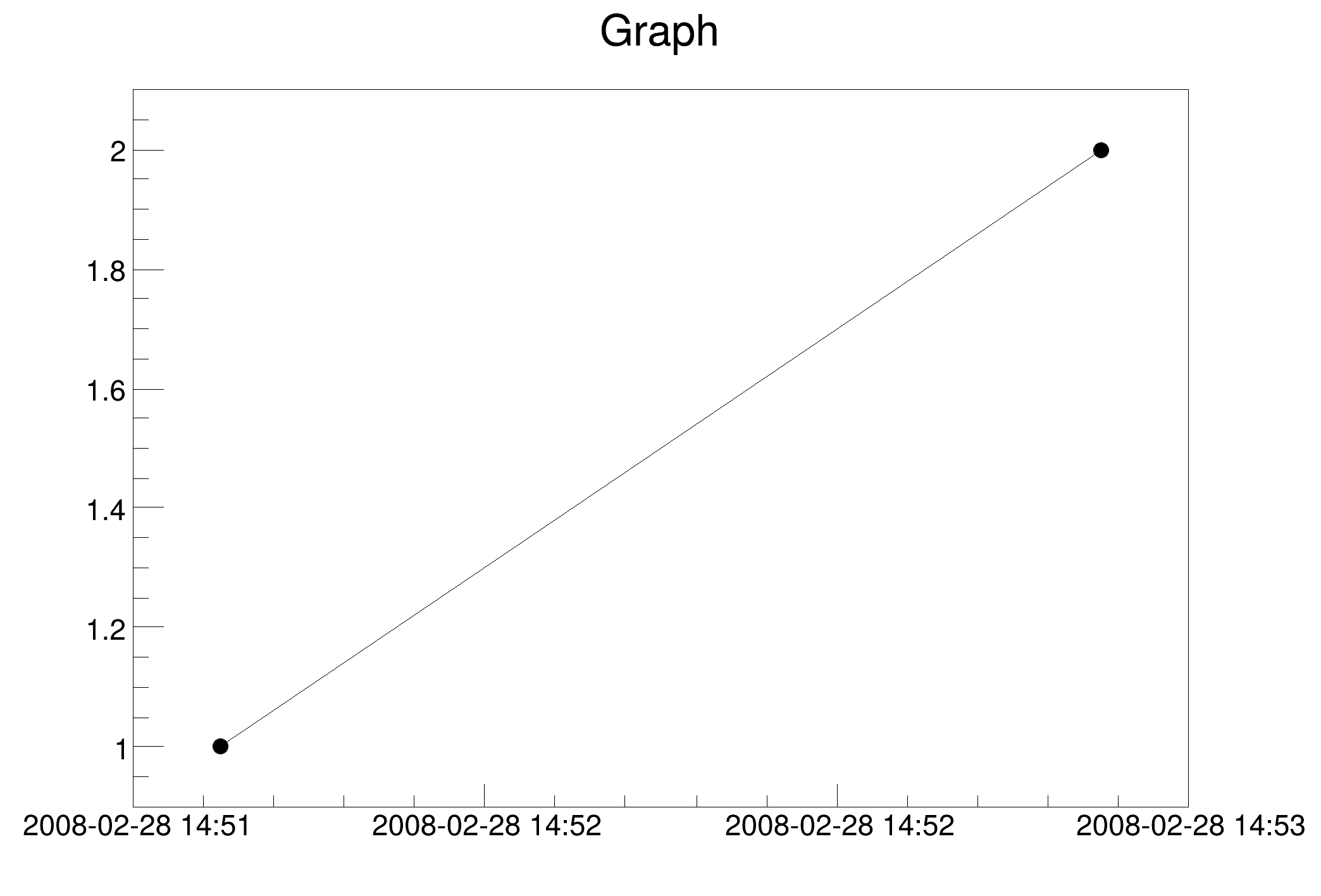The following example compares what the system time function gmtime and localtime give with what gives TGaxis. It can be used as referenced test to check if the time option of TGaxis is working properly.

#include "TAxis.h"
#include "TGaxis.h"
#include "TCanvas.h"
#include "TString.h"
#include "TLine.h"
#include "TLatex.h"
#include <time.h>
#include <stdio.h>
TString stime(time_t* t, bool utc = false, bool display_time_zone = true) {
struct tm* tt;
if (utc) tt = gmtime(t);
else tt = localtime(t);
char buf;
if (display_time_zone) strftime(buf, sizeof(buf), "%Y-%m-%d %H:%M:%S %Z", tt);
else strftime(buf, sizeof(buf), "%Y-%m-%d %H:%M:%S", tt);
return TString(buf);
}
TCanvas *timeonaxis3() {
double f = 1.8;
TCanvas* c = new TCanvas;
TLatex tex1;
tex1.SetNDC();
tex1.SetTextFont(102);
tex1.SetTextSize(0.055*f);
TLatex tex3;
tex3.SetNDC();
tex3.SetTextFont(102);
tex3.SetTextSize(0.07*f);
TLatex tex2;
tex2.SetNDC();
tex2.SetTextFont(102);
tex2.SetTextSize(0.07*f);
time_t offset[] = {0, 0, 1325376000, 1341100800};
time_t t[] = {1331150400, 1336417200, 0, 36000};
c->SetTopMargin(0); c->SetBottomMargin(0);
c->SetLeftMargin(0); c->SetRightMargin(0);
c->Divide(2, 4, -1, -1);
l.DrawLine(0.5, 0, 0.5, 1.);
for(int i = 0; i < 4; ++i){
for(int gmt = 0; gmt < 2; ++gmt){
const char* opt = (gmt ? "gmt" : "local");
TVirtualPad* p = c->cd(2*i + gmt + 1);
p->SetTopMargin(0); p->SetBottomMargin(0);
p->SetLeftMargin(0); p->SetRightMargin(0);
p->SetFillStyle(4000);
TGaxis* ga = new TGaxis (.4, .25, 5., .25, t[i], t[i] + 1, 1, "t");
ga->SetTimeFormat("TGaxis label: #color{%Y-%m-%d %H:%M:%S}");
ga->SetLabelFont(102);
ga->SetTimeOffset(offset[i], opt);
ga->SetLabelOffset(0.04*f);
ga->SetLabelSize(0.07*f);
ga->SetLineColor(0);
ga->Draw();
// Get offset string of axis time format: there is not accessor
// to time format in TGaxis.
// Assumes TAxis use the same format.
TAxis a(10, 0, 1600000000);
a.SetTimeOffset(offset[i], opt);
const char* offsettimeformat = a.GetTimeFormat();
char buf;
if (offset[i] < t[i]) {
sprintf(buf, "#splitline{%s, %s}{offset: %ld, option %s}",
stime(t+i).Data(), stime(t+i, true).Data(), offset[i], opt);
} else {
int h = t[i] / 3600;
int m = (t[i] - 3600 * h) / 60 ;
int s = (t[i] - h * 3600 - m * 60);
sprintf(buf, "#splitline{%d h %d m %d s}{offset: %s, option %s}",
h, m, s, stime(offset + i, gmt).Data(), opt);
}
tex1.DrawLatex(.01, .75, buf);
tex2.DrawLatex(.01, .50, offsettimeformat);
time_t t_ = t[i] + offset[i];
sprintf(buf, "Expecting: #color{%s}", stime(&t_, gmt, false).Data());
tex3.DrawLatex(.01, .24, buf);
if(i > 0) l.DrawLine(0, 0.95, 1, 0.95);
}
}
return c;
}
@ kOrange
Definition Rtypes.h:67
winID h TVirtualViewer3D TVirtualGLPainter p
Option_t Option_t TPoint TPoint const char GetTextMagnitude GetFillStyle GetLineColor GetLineWidth GetMarkerStyle GetTextAlign GetTextColor GetTextSize void char Point_t Rectangle_t WindowAttributes_t Float_t Float_t Float_t Int_t Int_t UInt_t UInt_t Rectangle_t Int_t Int_t Window_t TString Int_t GCValues_t GetPrimarySelectionOwner GetDisplay GetScreen GetColormap GetNativeEvent const char const char dpyName wid window const char font_name cursor keysym reg const char only_if_exist regb h Point_t winding char text const char depth char const char Int_t count const char ColorStruct_t color const char Pixmap_t Pixmap_t PictureAttributes_t attr const char char ret_data h unsigned char height h offset
virtual void SetTextColor(Color_t tcolor=1)
Set the text color.
Definition TAttText.h:44
virtual void SetTextFont(Font_t tfont=62)
Set the text font.
Definition TAttText.h:46
virtual void SetTextSize(Float_t tsize=1)
Set the text size.
Definition TAttText.h:47
void SetLabelFont(Int_t labelfont)
Definition TGaxis.h:105
void SetLabelColor(Int_t labelcolor)
Definition TGaxis.h:104
To draw Mathematical Formula.
Definition TLatex.h:18
TLatex * DrawLatex(Double_t x, Double_t y, const char *text)
Make a copy of this object with the new parameters And copy object attributes.
Definition TLatex.cxx:1928
Use the TLine constructor to create a simple line.
Definition TLine.h:22
virtual TLine * DrawLine(Double_t x1, Double_t y1, Double_t x2, Double_t y2)
Draw this line with new coordinates.
Definition TLine.cxx:103
Basic string class.
Definition TString.h:139
virtual void SetNDC(Bool_t isNDC=kTRUE)
Set NDC mode on if isNDC = kTRUE, off otherwise.
Definition TText.cxx:823
TVirtualPad is an abstract base class for the Pad and Canvas classes.
TMarker m
Definition textangle.C:8
TLine l
Definition textangle.C:4
auto * tt
Definition textangle.C:16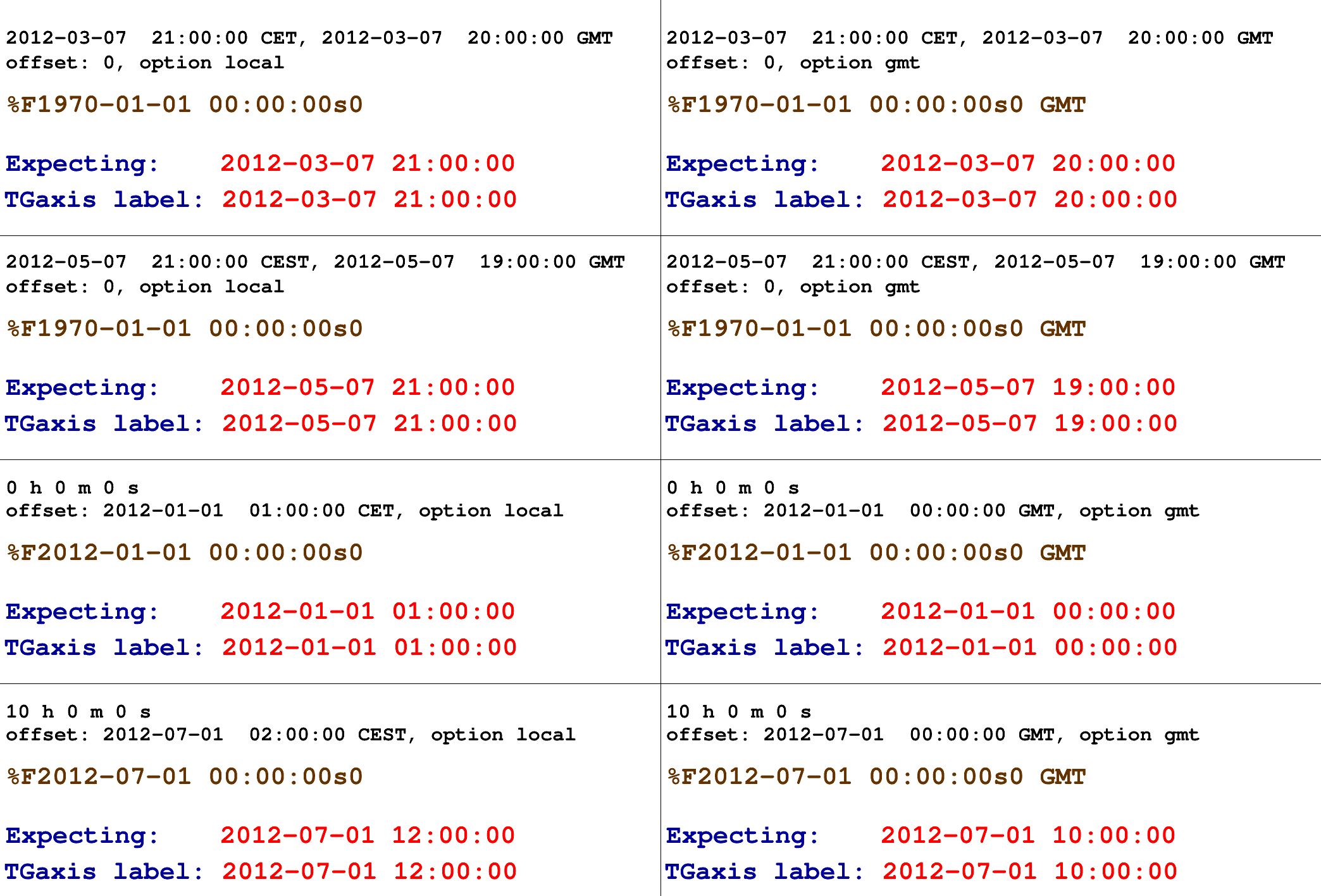The following macro illustrates the use, with histograms axis, of the time mode on the axis with different time intervals and time formats.

#include <time.h>
TCanvas *timeonaxis()
{
time_t script_time;
script_time = time(0);
script_time = 3600*(int)(script_time/3600);
// The time offset is the one that will be used by all graphs.
// If one changes it, it will be changed even on the graphs already defined
gStyle->SetTimeOffset(script_time);
TCanvas *ct = new TCanvas("ct","Time on axis",10,10,700,900);
ct->Divide(1,3);
int i;
//### Build a signal : noisy damped sine
// Time interval : 30 minutes
gStyle->SetTitleH(0.08);
float noise;
TH1F *ht = new TH1F("ht","Love at first sight",3000,0.,2000.);
for (i=1;i<3000;i++) {
noise = gRandom->Gaus(0,120);
if (i>700) {
noise += 1000*sin((i-700)*6.28/30)*exp((double)(700-i)/300);
}
ht->SetBinContent(i,noise);
}
ct->cd(1);
ht->SetLineColor(2);
ht->GetXaxis()->SetLabelSize(0.05);
ht->Draw();
// Sets time on the X axis
// The time used is the one set as time offset added to the value
// of the axis. This is converted into day/month/year hour:min:sec and
// a reasonable tick interval value is chosen.
//### Build a simple graph beginning at a different time
// Time interval : 5 seconds
float x, t;
for (i=0;i<100;i++) {
x[i] = sin(i*4*3.1415926/50)*exp(-(double)i/20);
t[i] = 6000+(double)i/20;
}
TGraph *gt = new TGraph(100,t,x);
gt->SetTitle("Politics");
ct->cd(2);
gt->SetLineColor(5);
gt->SetLineWidth(2);
gt->Draw("AL");
gt->GetXaxis()->SetLabelSize(0.05);
// Sets time on the X axis
//### Build a second simple graph for a very long time interval
// Time interval : a few years
float x2, t2;
for (i=0;i<10;i++) {
x2[i] = gRandom->Gaus(500,100)*i;
t2[i] = i*365*86400;
}
TGraph *gt2 = new TGraph(10,t2,x2);
gt2->SetTitle("Number of monkeys on the moon");
ct->cd(3);
gt2->SetMarkerColor(4);
gt2->SetMarkerStyle(29);
gt2->SetMarkerSize(1.3);
gt2->Draw("AP");
gt2->GetXaxis()->SetLabelSize(0.05);
// Sets time on the X axis
//
// One can choose a different time format than the one chosen by default
// The time format is the same as the one of the C strftime() function
// It's a string containing the following formats :
//
// for date :
// %a abbreviated weekday name
// %b abbreviated month name
// %d day of the month (01-31)
// %m month (01-12)
// %y year without century
// %Y year with century
//
// for time :
// %H hour (24-hour clock)
// %I hour (12-hour clock)
// %p local equivalent of AM or PM
// %M minute (00-59)
// %S seconds (00-61)
// %% %
// The other characters are output as is.
gt2->GetXaxis()->SetTimeFormat("y. %Y %F2000-01-01 00:00:00");
return ct;
}
virtual void SetLineWidth(Width_t lwidth)
Set the line width.
Definition TAttLine.h:43
virtual void SetMarkerColor(Color_t mcolor=1)
Set the marker color.
Definition TAttMarker.h:38
virtual void SetMarkerStyle(Style_t mstyle=1)
Set the marker style.
Definition TAttMarker.h:40
virtual void SetMarkerSize(Size_t msize=1)
Set the marker size.
Definition TAttMarker.h:45
Definition TCanvas.cxx:716
void Draw(Option_t *chopt="") override
Draw this graph with its current attributes.
Definition TGraph.cxx:809
TAxis * GetXaxis() const
Get x axis of the graph.
Definition TGraph.cxx:1540
void SetTitle(const char *title="") override
Change (i.e.
Definition TGraph.cxx:2370
virtual void SetBinContent(Int_t bin, Double_t content)
Set bin content see convention for numbering bins in TH1::GetBin In case the bin number is greater th...
Definition TH1.cxx:9091
void Divide(Int_t nx=1, Int_t ny=1, Float_t xmargin=0.01, Float_t ymargin=0.01, Int_t color=0) override
RVec< PromoteType< T > > exp(const RVec< T > &v)
Definition RVec.hxx:1800
RVec< PromoteType< T > > sin(const RVec< T > &v)
Definition RVec.hxx:1814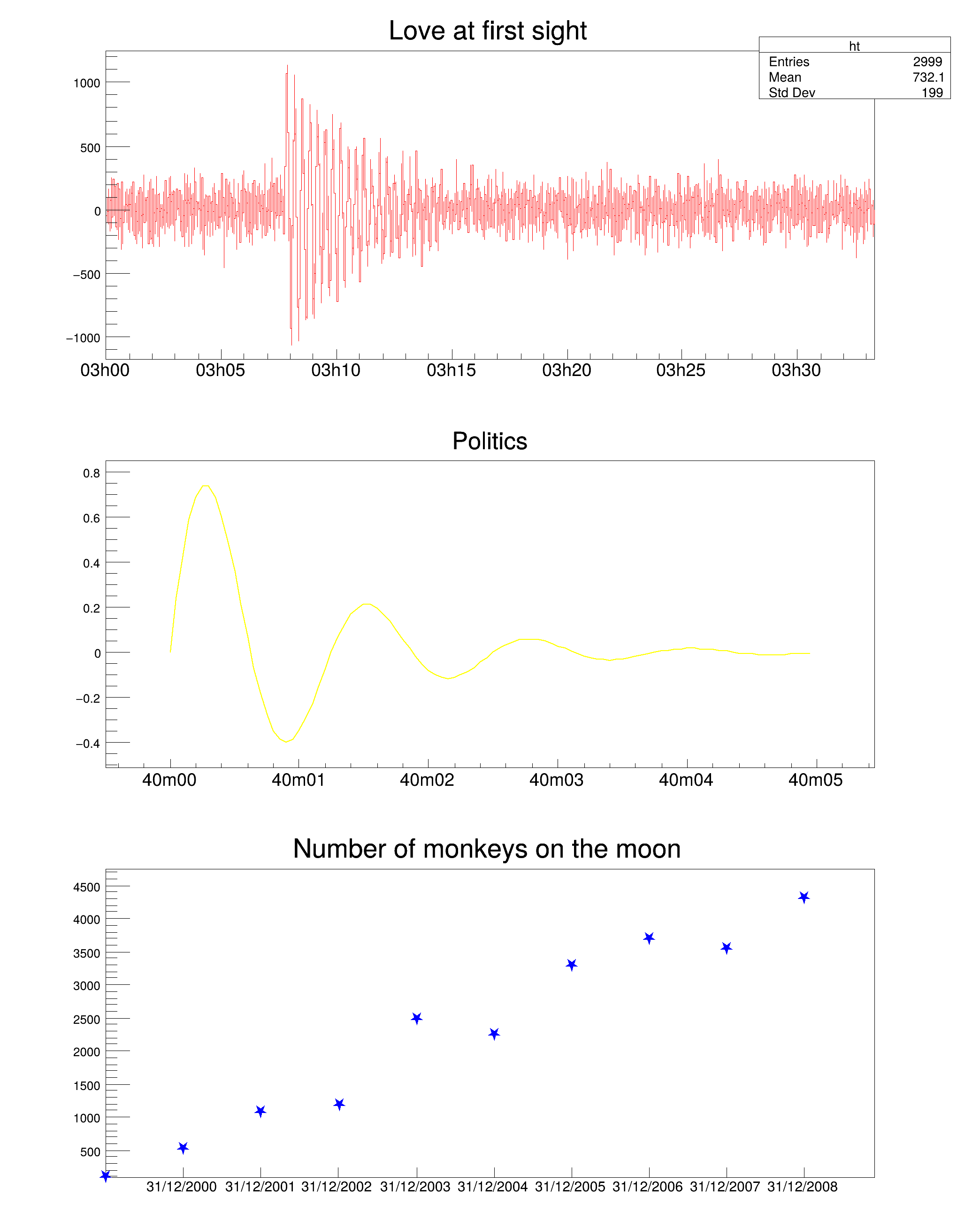Definition at line 24 of file TGaxis.h.

## Public Member Functions

TGaxis ()
TGaxis default constructor.

TGaxis (Double_t xmin, Double_t ymin, Double_t xmax, Double_t ymax, const char *funcname, Int_t ndiv=510, Option_t *chopt="", Double_t gridlength=0)
Constructor with a TF1 to map axis values.

TGaxis (Double_t xmin, Double_t ymin, Double_t xmax, Double_t ymax, Double_t wmin, Double_t wmax, Int_t ndiv=510, Option_t *chopt="", Double_t gridlength=0)
TGaxis normal constructor.

~TGaxis () override
TGaxis default destructor.

virtual void AdjustBinSize (Double_t A1, Double_t A2, Int_t nold, Double_t &BinLow, Double_t &BinHigh, Int_t &nbins, Double_t &BinWidth)
Internal method for axis labels optimisation.

virtual void CenterLabels (Bool_t center=kTRUE)
If center = kTRUE axis labels are centered in the center of the bin.

virtual void CenterTitle (Bool_t center=kTRUE)
If center = kTRUE axis title will be centered. The default is right adjusted.

void ChangeLabel (Int_t labNum=0, Double_t labAngle=-1., Double_t labSize=-1., Int_t labAlign=-1, Int_t labColor=-1, Int_t labFont=-1, const TString &labText="")
Define new text attributes for the label number "labNum".

void ChangeLabelAttributes (Int_t i, Int_t nlabels, TLatex *t, char *c, Double_t value=0., Double_t eps=-1.)

void ChangeLabelByValue (Double_t labValue, Double_t labAngle=-1., Double_t labSize=-1., Int_t labAlign=-1, Int_t labColor=-1, Int_t labFont=-1, const TString &labText="")

virtual TGaxisDrawAxis (Double_t xmin, Double_t ymin, Double_t xmax, Double_t ymax, Double_t wmin, Double_t wmax, Int_t ndiv=510, Option_t *chopt="", Double_t gridlength=0)
Draw this axis with new attributes.

TF1GetFunction () const

Float_t GetGridLength () const

Int_t GetLabelColor () const

Int_t GetLabelFont () const

Float_t GetLabelOffset () const

Float_t GetLabelSize () const

const char * GetName () const override
Returns name of object.

Int_t GetNdiv () const

const char * GetOption () const override

Float_t GetTickSize () const

const char * GetTitle () const override
Returns title of object.

Float_t GetTitleOffset () const

Float_t GetTitleSize () const

Double_t GetWmax () const

Double_t GetWmin () const

virtual void ImportAxisAttributes (TAxis *axis)
Internal method to import TAxis attributes to this TGaxis.

TClassIsA () const override

void LabelsLimits (const char *label, Int_t &first, Int_t &last)
Internal method to find first and last character of a label.

void Paint (Option_t *chopt="") override
Draw this axis with its current attributes.

virtual void PaintAxis (Double_t xmin, Double_t ymin, Double_t xmax, Double_t ymax, Double_t &wmin, Double_t &wmax, Int_t &ndiv, Option_t *chopt="", Double_t gridlength=0, Bool_t drawGridOnly=kFALSE)
Control function to draw an axis.

void ResetLabelAttributes (TLatex *t)

virtual void Rotate (Double_t X, Double_t Y, Double_t CFI, Double_t SFI, Double_t XT, Double_t YT, Double_t &U, Double_t &V)
Internal method to rotate axis coordinates.

void SavePrimitive (std::ostream &out, Option_t *option="") override
Save primitive as a C++ statement(s) on output stream out.

virtual void SetDecimals (Bool_t dot=kTRUE)
Set the decimals flag.

void SetFunction (const char *funcname="")
Specify a function to map the axis values.

void SetGridLength (Float_t gridlength)

void SetLabelColor (Int_t labelcolor)

void SetLabelFont (Int_t labelfont)

void SetLabelOffset (Float_t labeloffset)

void SetLabelSize (Float_t labelsize)

virtual void SetMoreLogLabels (Bool_t more=kTRUE)

virtual void SetName (const char *name)

virtual void SetNdivisions (Int_t ndiv)

virtual void SetNoExponent (Bool_t noExponent=kTRUE)

void SetOption (Option_t *option="")

void SetTickLength (Float_t ticklength)

void SetTickSize (Float_t ticksize)

void SetTimeFormat (const char *tformat)

void SetTimeOffset (Double_t toffset, Option_t *option="local")

virtual void SetTitle (const char *title="")

void SetTitleColor (Int_t titlecolor)

void SetTitleFont (Int_t titlefont)

void SetTitleOffset (Float_t titleoffset=1)

void SetTitleSize (Float_t titlesize)

void SetWmax (Double_t wmax)

void SetWmin (Double_t wmin)

void Streamer (TBuffer &) override
Stream an object of class TObject.

void StreamerNVirtual (TBuffer &ClassDef_StreamerNVirtual_b)Public Member Functions inherited from TLine
TLine ()

TLine (const TLine &line)
Line copy constructor.

TLine (Double_t x1, Double_t y1, Double_t x2, Double_t y2)
Line normal constructor.

~TLine () override=default

void Copy (TObject &line) const override
Copy this line to line.

Int_t DistancetoPrimitive (Int_t px, Int_t py) override
Compute distance from point px,py to a line.

virtual TLineDrawLine (Double_t x1, Double_t y1, Double_t x2, Double_t y2)
Draw this line with new coordinates.

virtual TLineDrawLineNDC (Double_t x1, Double_t y1, Double_t x2, Double_t y2)
Draw this line with new coordinates in NDC.

void ExecuteEvent (Int_t event, Int_t px, Int_t py) override
Execute action corresponding to one event.

Rectangle_t GetBBox () override
Return the bounding Box of the Line.

TPoint GetBBoxCenter () override
Return the center of the BoundingBox as TPoint in pixels.

Double_t GetX1 () const

Double_t GetX2 () const

Double_t GetY1 () const

Double_t GetY2 () const

TClassIsA () const override

Bool_t IsHorizontal ()
Check whether this line is to be drawn horizontally.

Bool_t IsVertical ()
Check whether this line is to be drawn vertically.

void ls (Option_t *option="") const override
List this line with its attributes.

TLineoperator= (const TLine &src)
Assignment operator.

void Paint (Option_t *option="") override
Paint this line with its current attributes.

virtual void PaintLine (Double_t x1, Double_t y1, Double_t x2, Double_t y2)
Draw this line with new coordinates.

virtual void PaintLineNDC (Double_t u1, Double_t v1, Double_t u2, Double_t v2)
Draw this line with new coordinates in NDC.

void Print (Option_t *option="") const override
Dump this line with its attributes.

void SavePrimitive (std::ostream &out, Option_t *option="") override
Save primitive as a C++ statement(s) on output stream out.

void SetBBoxCenter (const TPoint &p) override
Set center of the BoundingBox.

void SetBBoxCenterX (const Int_t x) override
Set X coordinate of the center of the BoundingBox.

void SetBBoxCenterY (const Int_t y) override
Set Y coordinate of the center of the BoundingBox.

void SetBBoxX1 (const Int_t x) override
Set left hand side of BoundingBox to a value (resize in x direction on left)

void SetBBoxX2 (const Int_t x) override
Set right hand side of BoundingBox to a value (resize in x direction on right)

void SetBBoxY1 (const Int_t y) override
Set top of BoundingBox to a value (resize in y direction on top)

void SetBBoxY2 (const Int_t y) override
Set bottom of BoundingBox to a value (resize in y direction on bottom)

void SetHorizontal (Bool_t set=kTRUE)
Force the line to be drawn horizontally.

virtual void SetNDC (Bool_t isNDC=kTRUE)
Set NDC mode on if isNDC = kTRUE, off otherwise.

void SetVertical (Bool_t set=kTRUE)
Force the line to be drawn vertically.

virtual void SetX1 (Double_t x1)

virtual void SetX2 (Double_t x2)

virtual void SetY1 (Double_t y1)

virtual void SetY2 (Double_t y2)

void Streamer (TBuffer &) override
Stream an object of class TLine.

void StreamerNVirtual (TBuffer &ClassDef_StreamerNVirtual_b)Public Member Functions inherited from TObject
TObject ()
TObject constructor.

TObject (const TObject &object)
TObject copy ctor.

virtual ~TObject ()
TObject destructor.

void AbstractMethod (const char *method) const
Use this method to implement an "abstract" method that you don't want to leave purely abstract.

Append graphics object to current pad.

virtual void Browse (TBrowser *b)
Browse object. May be overridden for another default action.

ULong_t CheckedHash ()
Check and record whether this class has a consistent Hash/RecursiveRemove setup (*) and then return the regular Hash value for this object.

virtual const char * ClassName () const
Returns name of class to which the object belongs.

virtual void Clear (Option_t *="")

virtual TObjectClone (const char *newname="") const
Make a clone of an object using the Streamer facility.

virtual Int_t Compare (const TObject *obj) const
Compare abstract method.

virtual void Delete (Option_t *option="")
Delete this object.

virtual void Draw (Option_t *option="")
Default Draw method for all objects.

virtual void DrawClass () const
Draw class inheritance tree of the class to which this object belongs.

virtual TObjectDrawClone (Option_t *option="") const
Draw a clone of this object in the current selected pad with: gROOT->SetSelectedPad(c1).

virtual void Dump () const
Dump contents of object on stdout.

virtual void Error (const char *method, const char *msgfmt,...) const
Issue error message.

virtual void Execute (const char *method, const char *params, Int_t *error=nullptr)
Execute method on this object with the given parameter string, e.g.

virtual void Execute (TMethod *method, TObjArray *params, Int_t *error=nullptr)
Execute method on this object with parameters stored in the TObjArray.

virtual void Fatal (const char *method, const char *msgfmt,...) const
Issue fatal error message.

virtual TObjectFindObject (const char *name) const
Must be redefined in derived classes.

virtual TObjectFindObject (const TObject *obj) const
Must be redefined in derived classes.

virtual Option_tGetDrawOption () const
Get option used by the graphics system to draw this object.

virtual const char * GetIconName () const
Returns mime type name of object.

virtual char * GetObjectInfo (Int_t px, Int_t py) const
Returns string containing info about the object at position (px,py).

virtual UInt_t GetUniqueID () const
Return the unique object id.

virtual Bool_t HandleTimer (TTimer *timer)
Execute action in response of a timer timing out.

virtual ULong_t Hash () const
Return hash value for this object.

Bool_t HasInconsistentHash () const
Return true is the type of this object is known to have an inconsistent setup for Hash and RecursiveRemove (i.e.

virtual void Info (const char *method, const char *msgfmt,...) const
Issue info message.

virtual Bool_t InheritsFrom (const char *classname) const
Returns kTRUE if object inherits from class "classname".

virtual Bool_t InheritsFrom (const TClass *cl) const
Returns kTRUE if object inherits from TClass cl.

virtual void Inspect () const
Dump contents of this object in a graphics canvas.

void InvertBit (UInt_t f)

Bool_t IsDestructed () const
IsDestructed.

virtual Bool_t IsEqual (const TObject *obj) const
Default equal comparison (objects are equal if they have the same address in memory).

virtual Bool_t IsFolder () const
Returns kTRUE in case object contains browsable objects (like containers or lists of other objects).

R__ALWAYS_INLINE Bool_t IsOnHeap () const

virtual Bool_t IsSortable () const

R__ALWAYS_INLINE Bool_t IsZombie () const

void MayNotUse (const char *method) const
Use this method to signal that a method (defined in a base class) may not be called in a derived class (in principle against good design since a child class should not provide less functionality than its parent, however, sometimes it is necessary).

virtual Bool_t Notify ()
This method must be overridden to handle object notification (the base implementation is no-op).

void Obsolete (const char *method, const char *asOfVers, const char *removedFromVers) const
Use this method to declare a method obsolete.

void operator delete (void *ptr)
Operator delete.

void operator delete[] (void *ptr)
Operator delete [].

void * operator new (size_t sz)

void * operator new (size_t sz, void *vp)

void * operator new[] (size_t sz)

void * operator new[] (size_t sz, void *vp)

TObjectoperator= (const TObject &rhs)
TObject assignment operator.

virtual void Pop ()
Pop on object drawn in a pad to the top of the display list.

virtual Int_t Read (const char *name)
Read contents of object with specified name from the current directory.

virtual void RecursiveRemove (TObject *obj)
Recursively remove this object from a list.

void ResetBit (UInt_t f)

virtual void SaveAs (const char *filename="", Option_t *option="") const
Save this object in the file specified by filename.

void SetBit (UInt_t f)

void SetBit (UInt_t f, Bool_t set)
Set or unset the user status bits as specified in f.

virtual void SetDrawOption (Option_t *option="")
Set drawing option for object.

virtual void SetUniqueID (UInt_t uid)
Set the unique object id.

void StreamerNVirtual (TBuffer &ClassDef_StreamerNVirtual_b)

virtual void SysError (const char *method, const char *msgfmt,...) const
Issue system error message.

R__ALWAYS_INLINE Bool_t TestBit (UInt_t f) const

Int_t TestBits (UInt_t f) const

virtual void UseCurrentStyle ()
Set current style settings in this object This function is called when either TCanvas::UseCurrentStyle or TROOT::ForceStyle have been invoked.

virtual void Warning (const char *method, const char *msgfmt,...) const
Issue warning message.

virtual Int_t Write (const char *name=nullptr, Int_t option=0, Int_t bufsize=0)
Write this object to the current directory.

virtual Int_t Write (const char *name=nullptr, Int_t option=0, Int_t bufsize=0) const
Write this object to the current directory.Public Member Functions inherited from TAttLine
TAttLine ()
AttLine default constructor.

TAttLine (Color_t lcolor, Style_t lstyle, Width_t lwidth)
AttLine normal constructor.

virtual ~TAttLine ()
AttLine destructor.

void Copy (TAttLine &attline) const
Copy this line attributes to a new TAttLine.

Int_t DistancetoLine (Int_t px, Int_t py, Double_t xp1, Double_t yp1, Double_t xp2, Double_t yp2)
Compute distance from point px,py to a line.

virtual Color_t GetLineColor () const
Return the line color.

virtual Style_t GetLineStyle () const
Return the line style.

virtual Width_t GetLineWidth () const
Return the line width.

virtual void Modify ()
Change current line attributes if necessary.

virtual void ResetAttLine (Option_t *option="")
Reset this line attributes to default values.

virtual void SaveLineAttributes (std::ostream &out, const char *name, Int_t coldef=1, Int_t stydef=1, Int_t widdef=1)
Save line attributes as C++ statement(s) on output stream out.

virtual void SetLineAttributes ()
Invoke the DialogCanvas Line attributes.

virtual void SetLineColor (Color_t lcolor)
Set the line color.

virtual void SetLineColorAlpha (Color_t lcolor, Float_t lalpha)
Set a transparent line color.

virtual void SetLineStyle (Style_t lstyle)
Set the line style.

virtual void SetLineWidth (Width_t lwidth)
Set the line width.

void StreamerNVirtual (TBuffer &ClassDef_StreamerNVirtual_b)Public Member Functions inherited from TAttBBox2D
virtual ~TAttBBox2D ()

void StreamerNVirtual (TBuffer &ClassDef_StreamerNVirtual_b)Public Member Functions inherited from TAttText
TAttText ()
AttText default constructor.

TAttText (Int_t align, Float_t angle, Color_t color, Style_t font, Float_t tsize)
AttText normal constructor.

virtual ~TAttText ()
AttText destructor.

void Copy (TAttText &atttext) const
Copy this text attributes to a new TAttText.

virtual Short_t GetTextAlign () const
Return the text alignment.

virtual Float_t GetTextAngle () const
Return the text angle.

virtual Color_t GetTextColor () const
Return the text color.

virtual Font_t GetTextFont () const
Return the text font.

virtual Float_t GetTextSize () const
Return the text size.

virtual Float_t GetTextSizePercent (Float_t size)
Return the text in percent of the pad size.

virtual void Modify ()
Change current text attributes if necessary.

virtual void ResetAttText (Option_t *toption="")
Reset this text attributes to default values.

virtual void SaveTextAttributes (std::ostream &out, const char *name, Int_t alidef=12, Float_t angdef=0, Int_t coldef=1, Int_t fondef=61, Float_t sizdef=1)
Save text attributes as C++ statement(s) on output stream out.

virtual void SetTextAlign (Short_t align=11)
Set the text alignment.

virtual void SetTextAngle (Float_t tangle=0)
Set the text angle.

virtual void SetTextAttributes ()
Invoke the DialogCanvas Text attributes.

virtual void SetTextColor (Color_t tcolor=1)
Set the text color.

virtual void SetTextColorAlpha (Color_t tcolor, Float_t talpha)
Set a transparent marker color.

virtual void SetTextFont (Font_t tfont=62)
Set the text font.

virtual void SetTextSize (Float_t tsize=1)
Set the text size.

virtual void SetTextSizePixels (Int_t npixels)
Set the text size in pixel.

void StreamerNVirtual (TBuffer &ClassDef_StreamerNVirtual_b)

## Static Public Member Functions

static TClassClass ()

static const char * Class_Name ()

static constexpr Version_t Class_Version ()

static const char * DeclFileName ()

static Int_t GetMaxDigits ()
Static function returning gStyle->GetAxisMaxDigits().

static void SetExponentOffset (Float_t xoff=0., Float_t yoff=0., Option_t *axis="xy")

static void SetMaxDigits (Int_t maxd=5)Static Public Member Functions inherited from TLine
static TClassClass ()

static const char * Class_Name ()

static constexpr Version_t Class_Version ()

static const char * DeclFileName ()Static Public Member Functions inherited from TObject
static TClassClass ()

static const char * Class_Name ()

static constexpr Version_t Class_Version ()

static const char * DeclFileName ()

static Longptr_t GetDtorOnly ()
Return destructor only flag.

static Bool_t GetObjectStat ()
Get status of object stat flag.

static void SetDtorOnly (void *obj)
Set destructor only flag.

static void SetObjectStat (Bool_t stat)
Turn on/off tracking of objects in the TObjectTable.Static Public Member Functions inherited from TAttLine
static TClassClass ()

static const char * Class_Name ()

static constexpr Version_t Class_Version ()

static const char * DeclFileName ()Static Public Member Functions inherited from TAttBBox2D
static TClassClass ()

static const char * Class_Name ()

static constexpr Version_t Class_Version ()

static const char * DeclFileName ()Static Public Member Functions inherited from TAttText
static TClassClass ()

static const char * Class_Name ()

static constexpr Version_t Class_Version ()

static const char * DeclFileName ()

## Protected Member Functions

TGaxis (const TGaxis &)
Copy constructor.

void CleanupModLabs ()
Correctly cleanup fModLabs - delete content when owned by TGaxis.

TAxisModLabFindModLab (Int_t indx, Int_t numlabels=0, Double_t v=0., Double_t eps=-1.) const
Search for axis modifier by index or value.

Bool_t IsOwnedModLabs () const
Returns kTRUE when fModLabs owned by TGaxis and should be cleaned up.

TGaxisoperator= (const TGaxis &)
Assignment operator.Protected Member Functions inherited from TObject
virtual void DoError (int level, const char *location, const char *fmt, va_list va) const
Interface to ErrorHandler (protected).

void MakeZombie ()

## Protected Attributes

TAxisfAxis
! Pointer to original TAxis axis (if any)

TString fChopt
Axis options.

TF1fFunction
! Pointer to function computing axis values

TString fFunctionName
Name of mapping function pointed by fFunction.

Float_t fGridLength
Length of the grid in NDC.

Int_t fLabelColor
Color for labels.

Int_t fLabelFont
Font for labels.

Float_t fLabelOffset
Offset of label wrt axis.

Float_t fLabelSize
Size of labels in NDC.

TListfModLabs
List of modified labels.

TString fName
Axis name.

Int_t fNdiv
Number of divisions.

Int_t fNModLabs
Number of modified labels.

Float_t fTickSize
Size of primary tick mark in NDC.

TString fTimeFormat
Time format, ex: 09/12/99 12:34:00.

TString fTitle
Axis title.

Float_t fTitleOffset
Offset of title wrt axis.

Float_t fTitleSize
Size of title in NDC.

Double_t fWmax
Highest value on the axis.

Double_t fWmin
Lowest value on the axis.Protected Attributes inherited from TLine
Double_t fX1 {0}
X of 1st point.

Double_t fX2 {0}
X of 2nd point.

Double_t fY1 {0}
Y of 1st point.

Double_t fY2 {0}
Y of 2nd point.Protected Attributes inherited from TAttLine
Color_t fLineColor
Line color.

Style_t fLineStyle
Line style.

Width_t fLineWidth
Line width.Protected Attributes inherited from TAttText
Short_t fTextAlign
Text alignment.

Float_t fTextAngle
Text angle.

Color_t fTextColor
Text color.

Font_t fTextFont
Text font.

Float_t fTextSize
Text size.Public Types inherited from TLine
enum  { kLineNDC = (1ULL << ( 14 )) , kVertical = (1ULL << ( 15 )) , kHorizontal = (1ULL << ( 16 )) }Public Types inherited from TObject
enum  {
kIsOnHeap = 0x01000000 , kNotDeleted = 0x02000000 , kZombie = 0x04000000 , kInconsistent = 0x08000000 ,
}

enum  { kSingleKey = (1ULL << ( 0 )) , kOverwrite = (1ULL << ( 1 )) , kWriteDelete = (1ULL << ( 2 )) }

enum  EDeprecatedStatusBits { kObjInCanvas = (1ULL << ( 3 )) }

enum  EStatusBits {
kCanDelete = (1ULL << ( 0 )) , kMustCleanup = (1ULL << ( 3 )) , kIsReferenced = (1ULL << ( 4 )) , kHasUUID = (1ULL << ( 5 )) ,
kCannotPick = (1ULL << ( 6 )) , kNoContextMenu = (1ULL << ( 8 )) , kInvalidObject = (1ULL << ( 13 ))
}Protected Types inherited from TObject
enum  { kOnlyPrepStep = (1ULL << ( 3 )) }

#include <TGaxis.h>

Inheritance diagram for TGaxis:
[legend]

## ◆ TGaxis() [1/4]

 TGaxis::TGaxis ( const TGaxis & ax )
protected

Copy constructor.

Definition at line 796 of file TGaxis.cxx.

## ◆ TGaxis() [2/4]

 TGaxis::TGaxis ( )

TGaxis default constructor.

Definition at line 694 of file TGaxis.cxx.

## ◆ TGaxis() [3/4]

 TGaxis::TGaxis ( Double_t xmin, Double_t ymin, Double_t xmax, Double_t ymax, Double_t wmin, Double_t wmax, Int_t ndiv = 510, Option_t * chopt = "", Double_t gridlength = 0 )

TGaxis normal constructor.

Definition at line 722 of file TGaxis.cxx.

## ◆ TGaxis() [4/4]

 TGaxis::TGaxis ( Double_t xmin, Double_t ymin, Double_t xmax, Double_t ymax, const char * funcname, Int_t ndiv = 510, Option_t * chopt = "", Double_t gridlength = 0 )

Constructor with a TF1 to map axis values.

Note
The function func (with name funcname) is not defined in the user's coordinate space, but in the new TGaxis space. If x is the original axis, w the new axis, and w = f(x) (for example, f is a calibration function converting ADC channels x to energy w), then func must be supplied as f^{-1}(w).

Definition at line 759 of file TGaxis.cxx.

## ◆ ~TGaxis()

 TGaxis::~TGaxis ( )
override

TGaxis default destructor.

Definition at line 861 of file TGaxis.cxx.

## Member Function Documentation

 void TGaxis::AdjustBinSize ( Double_t A1, Double_t A2, Int_t nold, Double_t & binLow, Double_t & binHigh, Int_t & nbins, Double_t & binWidth )
virtual

Internal method for axis labels optimisation.

This method adjusts the bining of the axis in order to have integer values for the labels.

Parameters
 [in] A1,A2 Old WMIN,WMAX [out] binLow,binHigh New WMIN,WMAX [in] nold Old NDIV (primary divisions) [out] nbins New NDIV [out] binWidth Bin width

Definition at line 2498 of file TGaxis.cxx.

## ◆ CenterLabels()

 void TGaxis::CenterLabels ( Bool_t center = kTRUE )
virtual

If center = kTRUE axis labels are centered in the center of the bin.

The default is to center on the primary tick marks. This option does not make sense if there are more bins than tick marks.

Definition at line 896 of file TGaxis.cxx.

## ◆ CenterTitle()

 void TGaxis::CenterTitle ( Bool_t center = kTRUE )
virtual

If center = kTRUE axis title will be centered. The default is right adjusted.

Definition at line 906 of file TGaxis.cxx.

## ◆ ChangeLabel()

 void TGaxis::ChangeLabel ( Int_t labNum = 0, Double_t labAngle = -1., Double_t labSize = -1., Int_t labAlign = -1, Int_t labColor = -1, Int_t labFont = -1, const TString & labText = "" )

Define new text attributes for the label number "labNum".

It allows to do a fine tuning of the labels. All the attributes can be changed, even the label text itself.

Parameters
 [in] labNum Number of the label to be changed, negative numbers start from the end [in] labAngle New angle value [in] labSize New size (0 erase the label) [in] labAlign New alignment value [in] labColor New label color [in] labFont New label font [in] labText New label text

#### Example:

{
auto c = new TCanvas("c1","Examples of TGaxis",900,100);
c->Range(-6,-0.1,6,0.1);
auto *axis = new TGaxis(-5.5,0.,5.5,0.,0.0,100,510,"S");
axis->SetName("axis1");
axis->SetTitle("Axis Title");
axis->SetTitleSize(0.2);
axis->SetLabelSize(0.2);
axis->SetTickSize(0.15);
axis->SetTitleColor(kBlue);
axis->SetTitleFont(42);
axis->ChangeLabel(1,-1,-1,-1,2);
axis->ChangeLabel(3,-1,0.);
axis->ChangeLabel(5,30.,-1,0);
axis->ChangeLabel(6,-1,-1,-1,3,-1,"6th label");
axis->ChangeLabel(-2,-1,-1,-1,3,-1,"2nd to last label");
axis->Draw();
}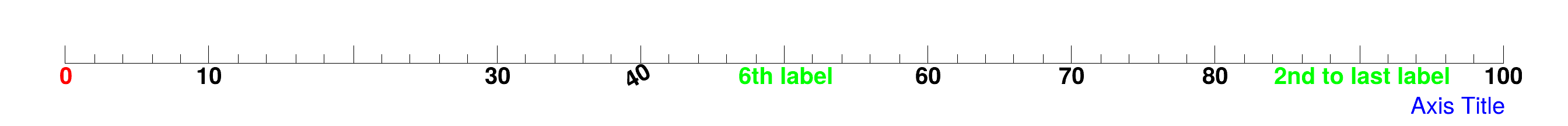#### Notes:

• If an attribute should not be changed just give the value "-1".
• If labnum=0 the list of modified labels is reset.
• To erase a label set labSize to 0.
• If labText is not specified or is an empty string, the text label is not changed.

Definition at line 2734 of file TGaxis.cxx.

## ◆ ChangeLabelAttributes()

 void TGaxis::ChangeLabelAttributes ( Int_t i, Int_t nlabels, TLatex * t, char * c, Double_t value = 0., Double_t eps = -1. )

## ◆ ChangeLabelByValue()

 void TGaxis::ChangeLabelByValue ( Double_t labValue, Double_t labAngle = -1., Double_t labSize = -1., Int_t labAlign = -1, Int_t labColor = -1, Int_t labFont = -1, const TString & labText = "" )

## ◆ Class()

 static TClass * TGaxis::Class ( )
static
Returns
TClass describing this class

## ◆ Class_Name()

 static const char * TGaxis::Class_Name ( )
static
Returns
Name of this class

## ◆ Class_Version()

 static constexpr Version_t TGaxis::Class_Version ( )
inlinestaticconstexpr
Returns
Version of this class

Definition at line 136 of file TGaxis.h.

## ◆ CleanupModLabs()

 void TGaxis::CleanupModLabs ( )
protected

Correctly cleanup fModLabs - delete content when owned by TGaxis.

Definition at line 881 of file TGaxis.cxx.

## ◆ DeclFileName()

 static const char * TGaxis::DeclFileName ( )
inlinestatic
Returns
Name of the file containing the class declaration

Definition at line 136 of file TGaxis.h.

## ◆ DrawAxis()

 TGaxis * TGaxis::DrawAxis ( Double_t xmin, Double_t ymin, Double_t xmax, Double_t ymax, Double_t wmin, Double_t wmax, Int_t ndiv = 510, Option_t * chopt = "", Double_t gridlength = 0 )
virtual

Draw this axis with new attributes.

Definition at line 916 of file TGaxis.cxx.

## ◆ FindModLab()

 TAxisModLab * TGaxis::FindModLab ( Int_t indx, Int_t numlabels = 0, Double_t v = 0., Double_t eps = -1. ) const
protected

Search for axis modifier by index or value.

Definition at line 2662 of file TGaxis.cxx.

## ◆ GetFunction()

 TF1 * TGaxis::GetFunction ( ) const
inline

Definition at line 77 of file TGaxis.h.

## ◆ GetGridLength()

 Float_t TGaxis::GetGridLength ( ) const
inline

Definition at line 76 of file TGaxis.h.

## ◆ GetLabelColor()

 Int_t TGaxis::GetLabelColor ( ) const
inline

Definition at line 78 of file TGaxis.h.

## ◆ GetLabelFont()

 Int_t TGaxis::GetLabelFont ( ) const
inline

Definition at line 79 of file TGaxis.h.

## ◆ GetLabelOffset()

 Float_t TGaxis::GetLabelOffset ( ) const
inline

Definition at line 80 of file TGaxis.h.

## ◆ GetLabelSize()

 Float_t TGaxis::GetLabelSize ( ) const
inline

Definition at line 81 of file TGaxis.h.

## ◆ GetMaxDigits()

 Int_t TGaxis::GetMaxDigits ( )
static

Static function returning gStyle->GetAxisMaxDigits().

Definition at line 947 of file TGaxis.cxx.

## ◆ GetName()

 const char * TGaxis::GetName ( ) const
inlineoverridevirtual

Returns name of object.

This default method returns the class name. Classes that give objects a name should override this method.

Reimplemented from TObject.

Definition at line 84 of file TGaxis.h.

## ◆ GetNdiv()

 Int_t TGaxis::GetNdiv ( ) const
inline

Definition at line 88 of file TGaxis.h.

## ◆ GetOption()

 const char * TGaxis::GetOption ( ) const
inlineoverridevirtual

Reimplemented from TObject.

Definition at line 85 of file TGaxis.h.

## ◆ GetTickSize()

 Float_t TGaxis::GetTickSize ( ) const
inline

Definition at line 91 of file TGaxis.h.

## ◆ GetTitle()

 const char * TGaxis::GetTitle ( ) const
inlineoverridevirtual

Returns title of object.

This default method returns the class title (i.e. description). Classes that give objects a title should override this method.

Reimplemented from TObject.

Definition at line 86 of file TGaxis.h.

## ◆ GetTitleOffset()

 Float_t TGaxis::GetTitleOffset ( ) const
inline

Definition at line 82 of file TGaxis.h.

## ◆ GetTitleSize()

 Float_t TGaxis::GetTitleSize ( ) const
inline

Definition at line 83 of file TGaxis.h.

## ◆ GetWmax()

 Double_t TGaxis::GetWmax ( ) const
inline

Definition at line 90 of file TGaxis.h.

## ◆ GetWmin()

 Double_t TGaxis::GetWmin ( ) const
inline

Definition at line 89 of file TGaxis.h.

## ◆ ImportAxisAttributes()

 void TGaxis::ImportAxisAttributes ( TAxis * axis )
virtual

Internal method to import TAxis attributes to this TGaxis.

Definition at line 955 of file TGaxis.cxx.

## ◆ IsA()

 TClass * TGaxis::IsA ( ) const
inlineoverridevirtual
Returns
TClass describing current object

Reimplemented from TObject.

Definition at line 136 of file TGaxis.h.

## ◆ IsOwnedModLabs()

 Bool_t TGaxis::IsOwnedModLabs ( ) const
protected

Returns kTRUE when fModLabs owned by TGaxis and should be cleaned up.

Definition at line 869 of file TGaxis.cxx.

## ◆ LabelsLimits()

 void TGaxis::LabelsLimits ( const char * label, Int_t & first, Int_t & last )

Internal method to find first and last character of a label.

Definition at line 2533 of file TGaxis.cxx.

## ◆ operator=()

 TGaxis & TGaxis::operator= ( const TGaxis & ax )
protected

Assignment operator.

Definition at line 828 of file TGaxis.cxx.

## ◆ Paint()

 void TGaxis::Paint ( Option_t * chopt = "" )
overridevirtual

Draw this axis with its current attributes.

Reimplemented from TObject.

Definition at line 986 of file TGaxis.cxx.

## ◆ PaintAxis()

 void TGaxis::PaintAxis ( Double_t xmin, Double_t ymin, Double_t xmax, Double_t ymax, Double_t & wmin, Double_t & wmax, Int_t & ndiv, Option_t * chopt = "", Double_t gridlength = 0, Bool_t drawGridOnly = kFALSE )
virtual

Control function to draw an axis.

Original authors: O.Couet C.E.Vandoni N.Cremel-Somon. Modified and converted to C++ class by Rene Brun.

Definition at line 1008 of file TGaxis.cxx.

## ◆ ResetLabelAttributes()

 void TGaxis::ResetLabelAttributes ( TLatex * t )

## ◆ Rotate()

 void TGaxis::Rotate ( Double_t X, Double_t Y, Double_t CFI, Double_t SFI, Double_t XT, Double_t YT, Double_t & U, Double_t & V )
virtual

Internal method to rotate axis coordinates.

Definition at line 2545 of file TGaxis.cxx.

## ◆ SavePrimitive()

 void TGaxis::SavePrimitive ( std::ostream & out, Option_t * option = "" )
overridevirtual

Save primitive as a C++ statement(s) on output stream out.

Reimplemented from TObject.

Definition at line 2555 of file TGaxis.cxx.

## ◆ SetDecimals()

 void TGaxis::SetDecimals ( Bool_t dot = kTRUE )
virtual

Set the decimals flag.

By default, blank characters are stripped, and then the label is correctly aligned. The dot, if last character of the string, is also stripped, unless this option is specified. One can disable the option by calling axis.SetDecimals(kTRUE). Note the bit is set in fBits (as opposed to fBits2 in TAxis!)

Definition at line 2634 of file TGaxis.cxx.

## ◆ SetExponentOffset()

 static void TGaxis::SetExponentOffset ( Float_t xoff = 0., Float_t yoff = 0., Option_t * axis = "xy" )
static

## ◆ SetFunction()

 void TGaxis::SetFunction ( const char * funcname = "" )

Specify a function to map the axis values.

Definition at line 2643 of file TGaxis.cxx.

## ◆ SetGridLength()

 void TGaxis::SetGridLength ( Float_t gridlength )
inline

Definition at line 124 of file TGaxis.h.

## ◆ SetLabelColor()

 void TGaxis::SetLabelColor ( Int_t labelcolor )
inline

Definition at line 104 of file TGaxis.h.

## ◆ SetLabelFont()

 void TGaxis::SetLabelFont ( Int_t labelfont )
inline

Definition at line 105 of file TGaxis.h.

## ◆ SetLabelOffset()

 void TGaxis::SetLabelOffset ( Float_t labeloffset )
inline

Definition at line 106 of file TGaxis.h.

## ◆ SetLabelSize()

 void TGaxis::SetLabelSize ( Float_t labelsize )
inline

Definition at line 107 of file TGaxis.h.

## ◆ SetMaxDigits()

 static void TGaxis::SetMaxDigits ( Int_t maxd = 5 )
static

## ◆ SetMoreLogLabels()

 virtual void TGaxis::SetMoreLogLabels ( Bool_t more = kTRUE )
virtual

## ◆ SetName()

 virtual void TGaxis::SetName ( const char * name )
virtual

## ◆ SetNdivisions()

 virtual void TGaxis::SetNdivisions ( Int_t ndiv )
inlinevirtual

Definition at line 118 of file TGaxis.h.

## ◆ SetNoExponent()

 virtual void TGaxis::SetNoExponent ( Bool_t noExponent = kTRUE )
virtual

## ◆ SetOption()

 void TGaxis::SetOption ( Option_t * option = "" )

## ◆ SetTickLength()

 void TGaxis::SetTickLength ( Float_t ticklength )
inline

Definition at line 123 of file TGaxis.h.

## ◆ SetTickSize()

 void TGaxis::SetTickSize ( Float_t ticksize )
inline

Definition at line 122 of file TGaxis.h.

## ◆ SetTimeFormat()

 void TGaxis::SetTimeFormat ( const char * tformat )

## ◆ SetTimeOffset()

 void TGaxis::SetTimeOffset ( Double_t toffset, Option_t * option = "local" )

## ◆ SetTitle()

 virtual void TGaxis::SetTitle ( const char * title = "" )
virtual

## ◆ SetTitleColor()

 void TGaxis::SetTitleColor ( Int_t titlecolor )
inline

Definition at line 131 of file TGaxis.h.

## ◆ SetTitleFont()

 void TGaxis::SetTitleFont ( Int_t titlefont )
inline

Definition at line 130 of file TGaxis.h.

## ◆ SetTitleOffset()

 void TGaxis::SetTitleOffset ( Float_t titleoffset = 1` )
inline

Definition at line 128 of file TGaxis.h.

## ◆ SetTitleSize()

 void TGaxis::SetTitleSize ( Float_t titlesize )
inline

Definition at line 129 of file TGaxis.h.

## ◆ SetWmax()

 void TGaxis::SetWmax ( Double_t wmax )
inline

Definition at line 133 of file TGaxis.h.

## ◆ SetWmin()

 void TGaxis::SetWmin ( Double_t wmin )
inline

Definition at line 132 of file TGaxis.h.

## ◆ Streamer()

 void TGaxis::Streamer ( TBuffer & R__b )
overridevirtual

Stream an object of class TObject.

Reimplemented from TObject.

## ◆ StreamerNVirtual()

 void TGaxis::StreamerNVirtual ( TBuffer & ClassDef_StreamerNVirtual_b )
inline

Definition at line 136 of file TGaxis.h.

## ◆ fAxis

 TAxis* TGaxis::fAxis
protected

! Pointer to original TAxis axis (if any)

Definition at line 46 of file TGaxis.h.

## ◆ fChopt

 TString TGaxis::fChopt
protected

Axis options.

Definition at line 40 of file TGaxis.h.

## ◆ fFunction

 TF1* TGaxis::fFunction
protected

! Pointer to function computing axis values

Definition at line 45 of file TGaxis.h.

## ◆ fFunctionName

 TString TGaxis::fFunctionName
protected

Name of mapping function pointed by fFunction.

Definition at line 44 of file TGaxis.h.

## ◆ fGridLength

 Float_t TGaxis::fGridLength
protected

Length of the grid in NDC.

Definition at line 30 of file TGaxis.h.

## ◆ fLabelColor

 Int_t TGaxis::fLabelColor
protected

Color for labels.

Definition at line 37 of file TGaxis.h.

## ◆ fLabelFont

 Int_t TGaxis::fLabelFont
protected

Font for labels.

Definition at line 38 of file TGaxis.h.

## ◆ fLabelOffset

 Float_t TGaxis::fLabelOffset
protected

Offset of label wrt axis.

Definition at line 32 of file TGaxis.h.

## ◆ fLabelSize

 Float_t TGaxis::fLabelSize
protected

Size of labels in NDC.

Definition at line 33 of file TGaxis.h.

## ◆ fModLabs

 TList* TGaxis::fModLabs
protected

List of modified labels.

Definition at line 47 of file TGaxis.h.

## ◆ fName

 TString TGaxis::fName
protected

Axis name.

Definition at line 41 of file TGaxis.h.

## ◆ fNdiv

 Int_t TGaxis::fNdiv
protected

Number of divisions.

Definition at line 36 of file TGaxis.h.

## ◆ fNModLabs

 Int_t TGaxis::fNModLabs
protected

Number of modified labels.

Definition at line 39 of file TGaxis.h.

## ◆ fTickSize

 Float_t TGaxis::fTickSize
protected

Size of primary tick mark in NDC.

Definition at line 31 of file TGaxis.h.

## ◆ fTimeFormat

 TString TGaxis::fTimeFormat
protected

Time format, ex: 09/12/99 12:34:00.

Definition at line 43 of file TGaxis.h.

## ◆ fTitle

 TString TGaxis::fTitle
protected

Axis title.

Definition at line 42 of file TGaxis.h.

## ◆ fTitleOffset

 Float_t TGaxis::fTitleOffset
protected

Offset of title wrt axis.

Definition at line 34 of file TGaxis.h.

## ◆ fTitleSize

 Float_t TGaxis::fTitleSize
protected

Size of title in NDC.

Definition at line 35 of file TGaxis.h.

## ◆ fWmax

 Double_t TGaxis::fWmax
protected

Highest value on the axis.

Definition at line 29 of file TGaxis.h.

## ◆ fWmin

 Double_t TGaxis::fWmin
protected

Lowest value on the axis.

Definition at line 28 of file TGaxis.h.

Libraries for TGaxis:[legend]

The documentation for this class was generated from the following files: